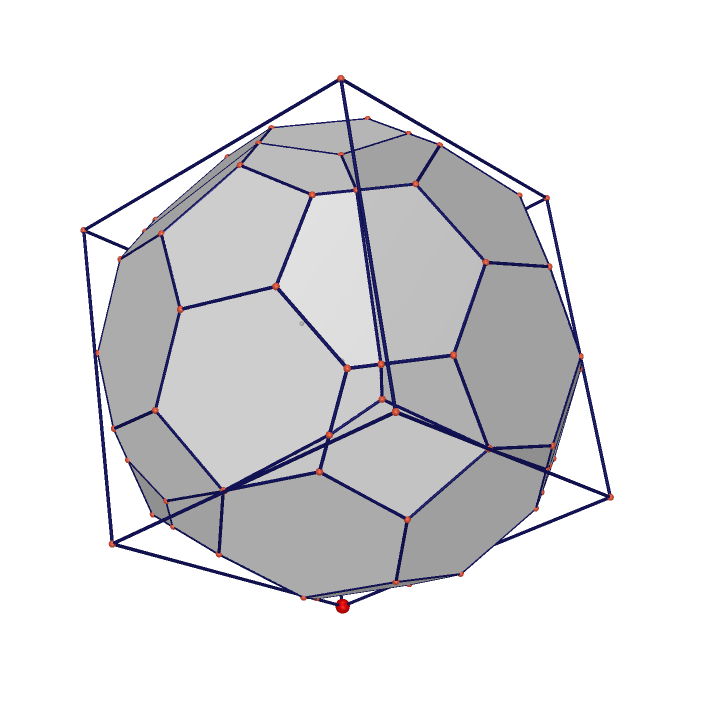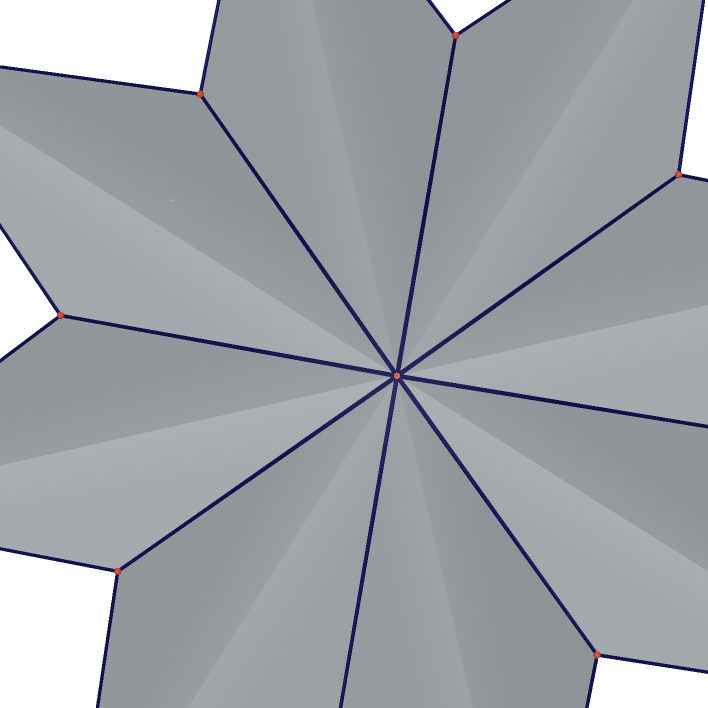101021218 °ĘşA´X¦ó

101021218 ĽĆľÇ16 ŞL«Ű§Ę

 Icosidodecahedron Cuboctahedron Buckyball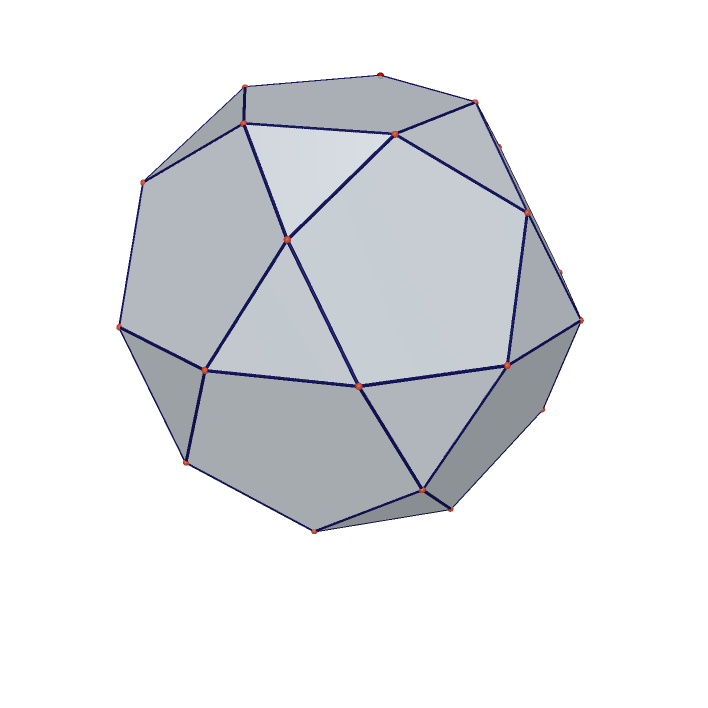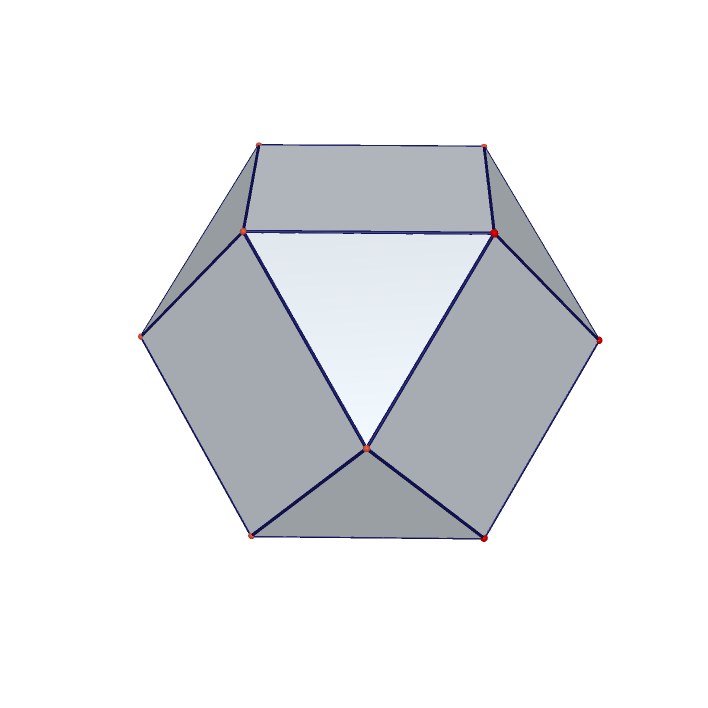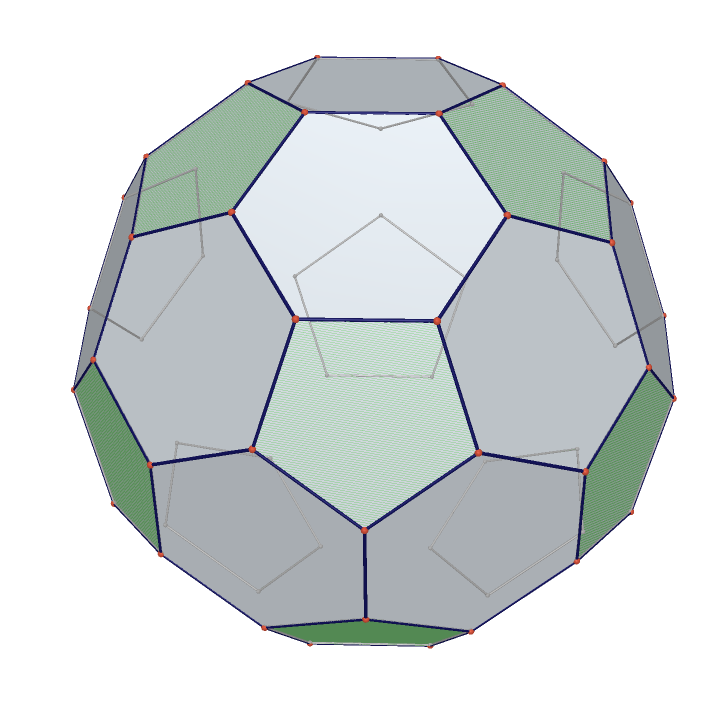Cube(Convex hull of Two Tetrahedrons) Rhombic dodecahedron(Convex hull of cube and octahedron) Rhombic triacontahedron(Convex hull of dodecahedron and icosahedron)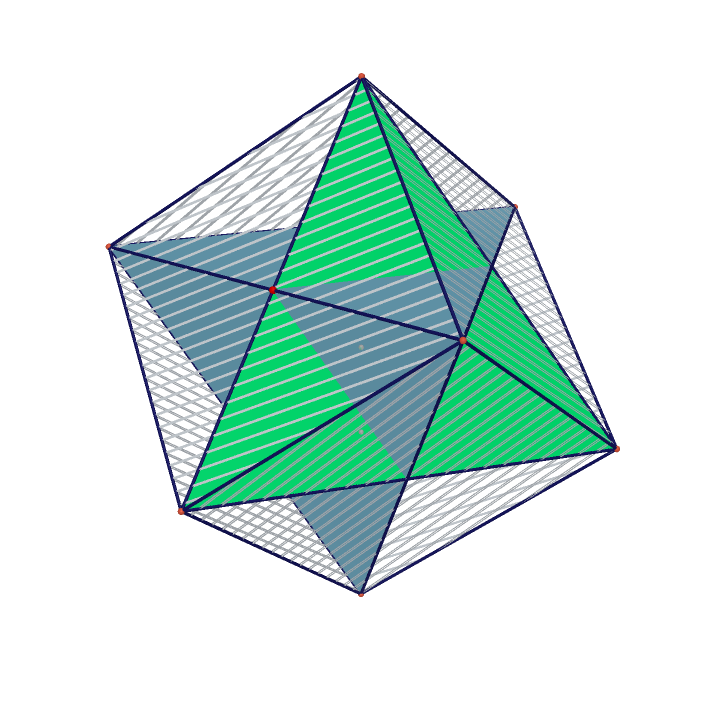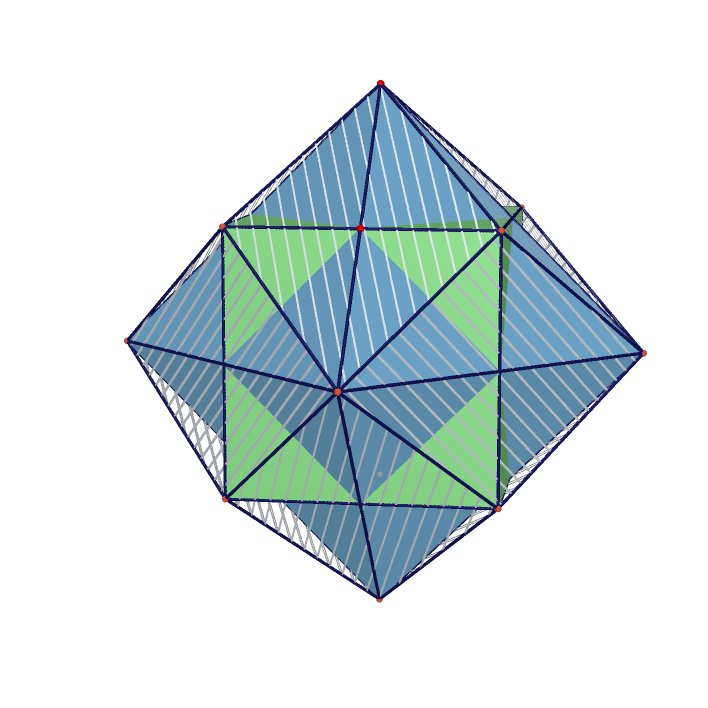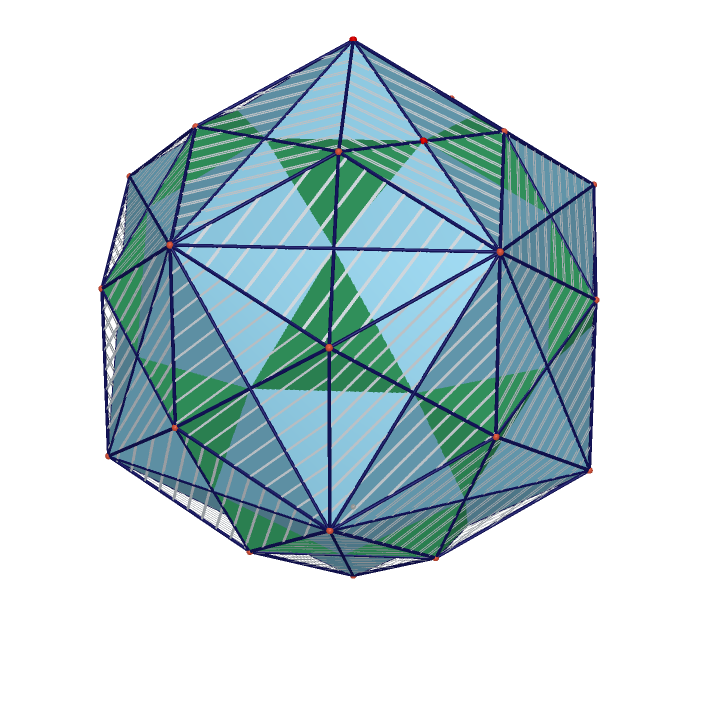Duality Between Cube and Octahedron Duality Between Dodecahedron and Icosahedron Duality Between Tetrahedron and Itself(Stellated octahedron)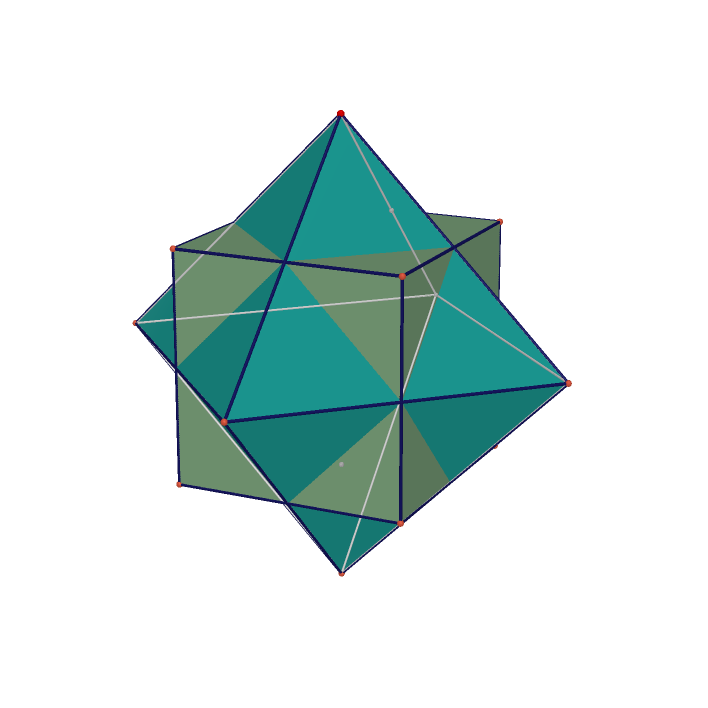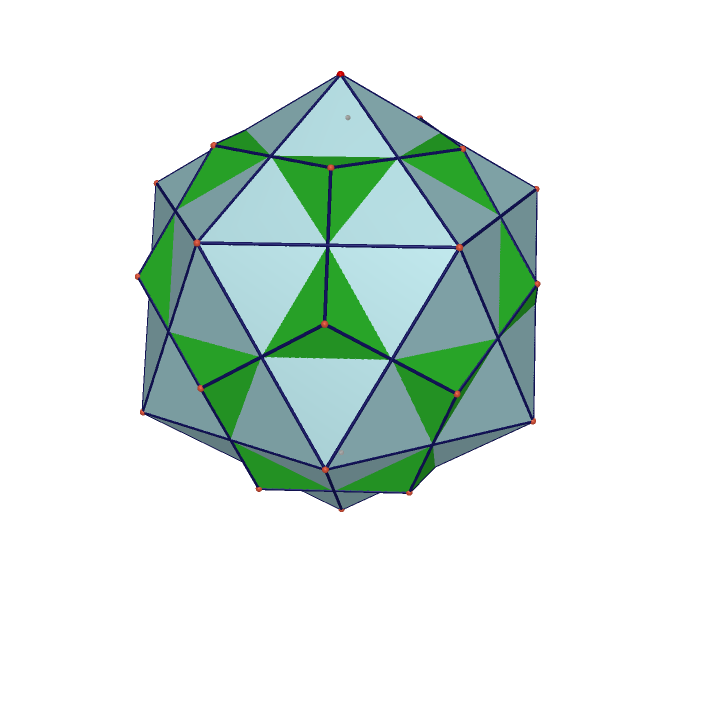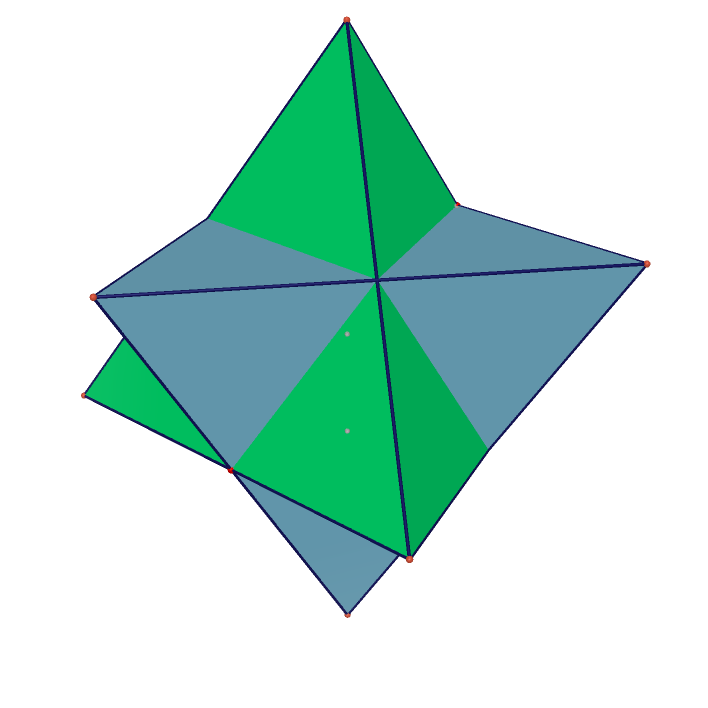Rhombic Icosahedron Moving Rhombic icosahedron Moving Rhombic 132 Faces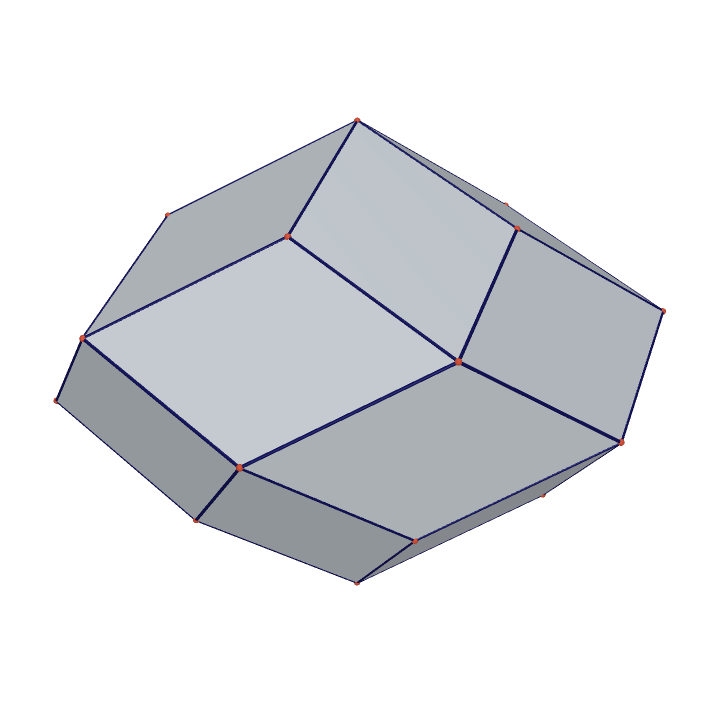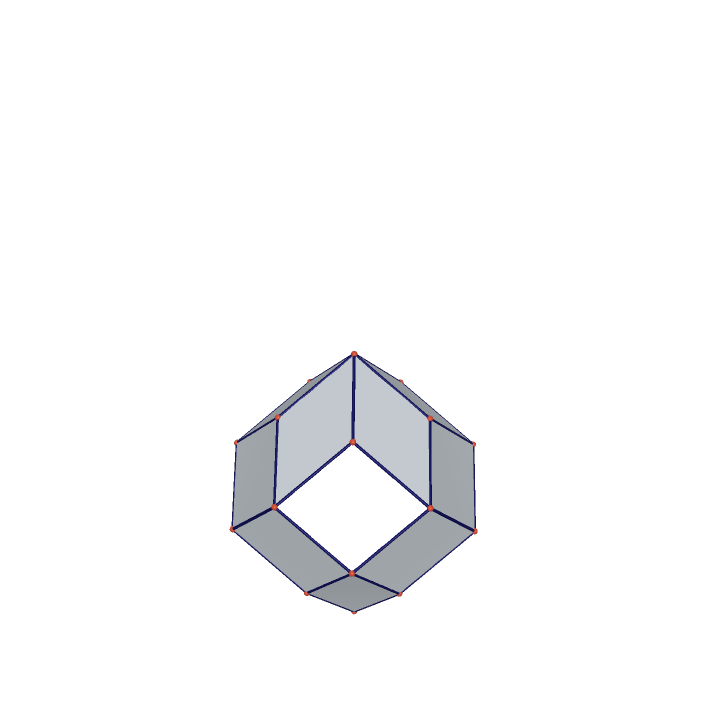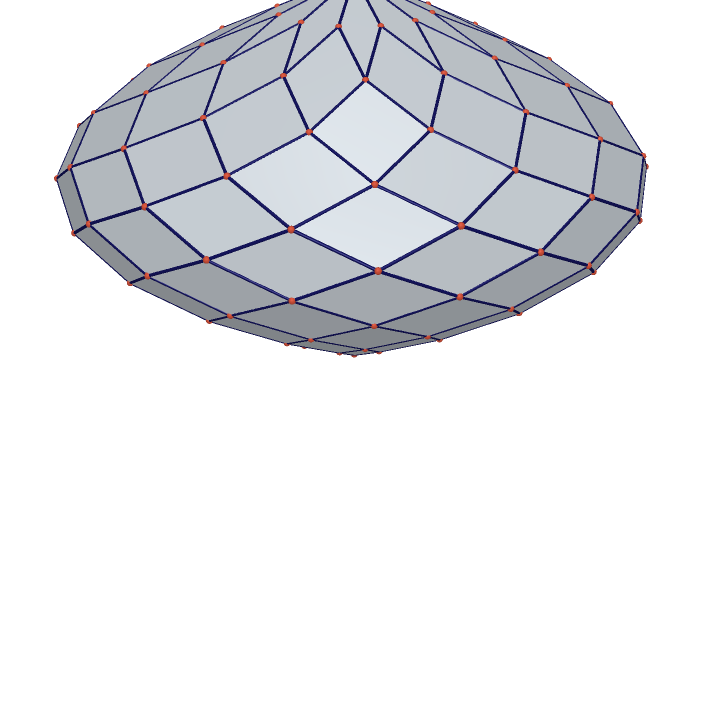Rhombic with 120 Faces Rhombic with 180 Faces Rhombic wirh 240 Faces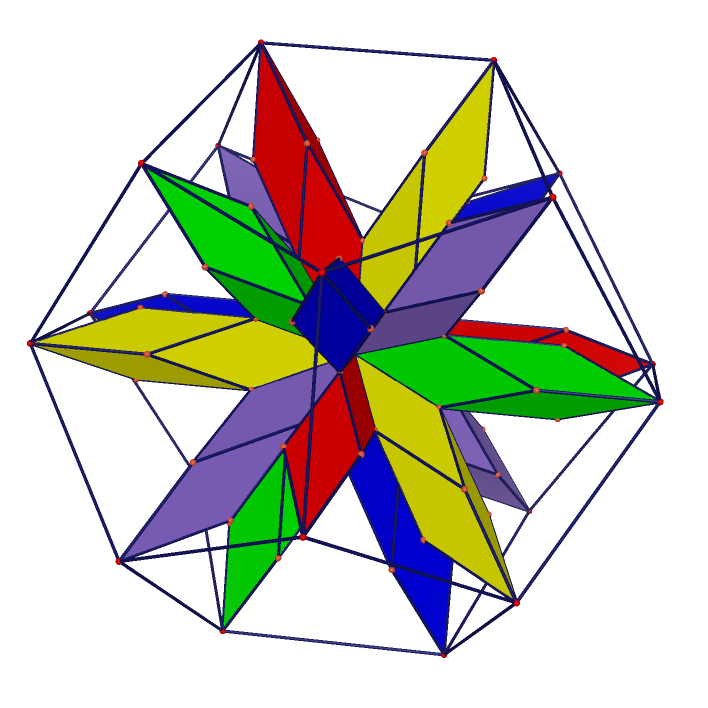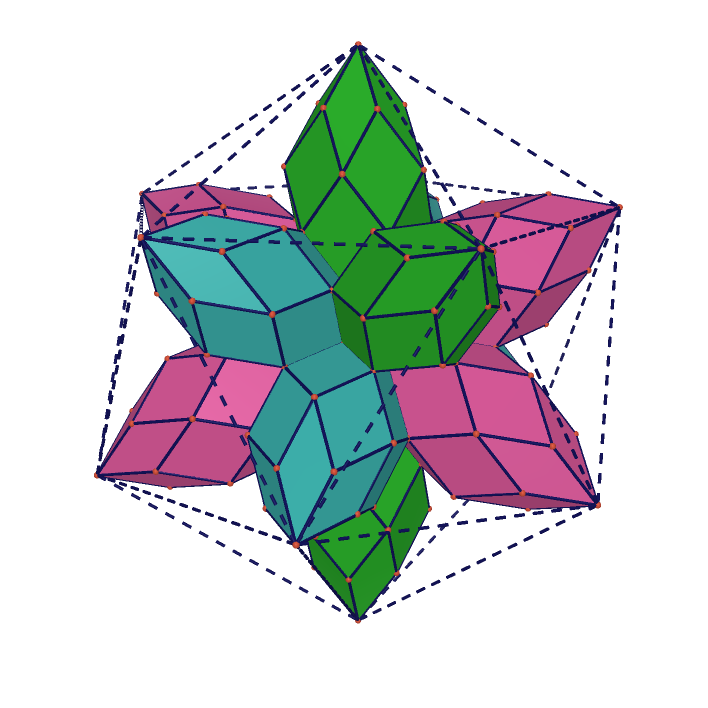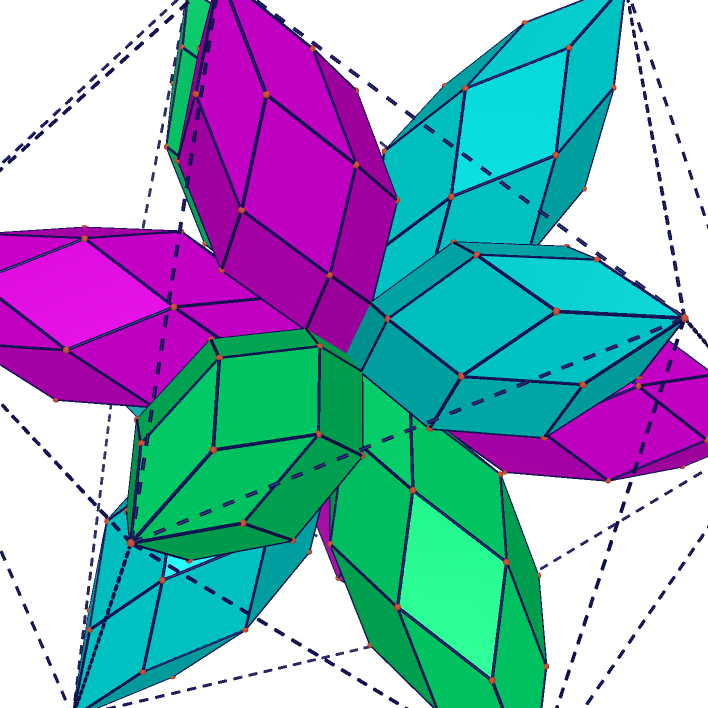Icosahedron in 5 tetrahedron in dodecahedron Intersection of 5 tetrahedrons(Chiro icosahedron) The intersection of 5 cubes(Rhombic triacontahedron)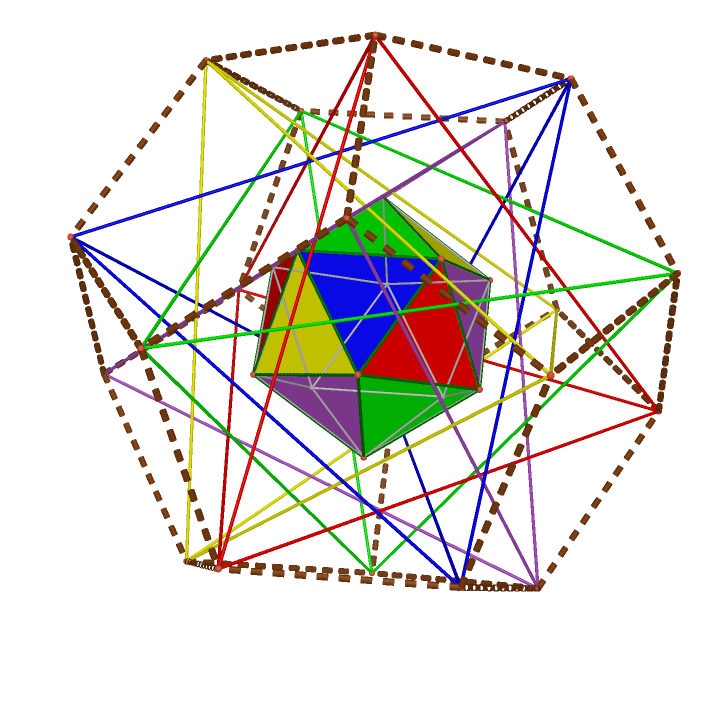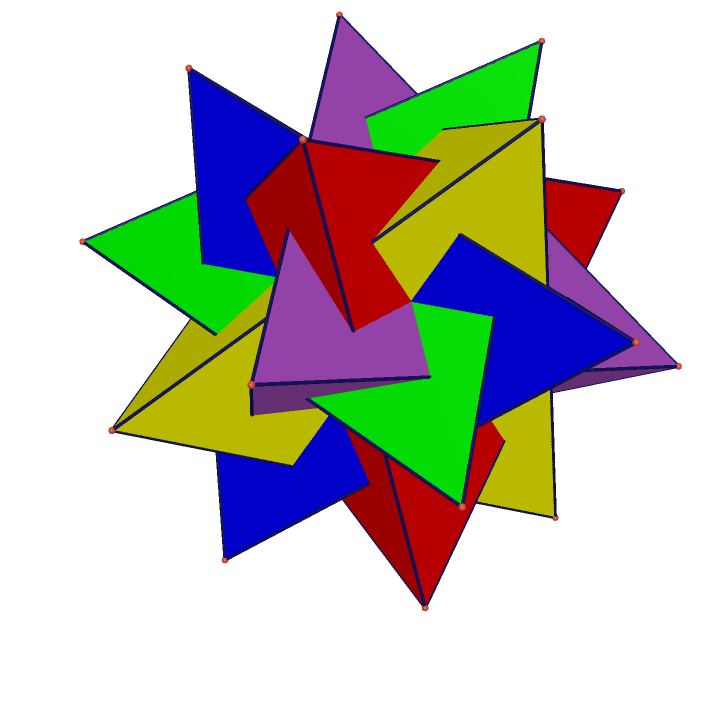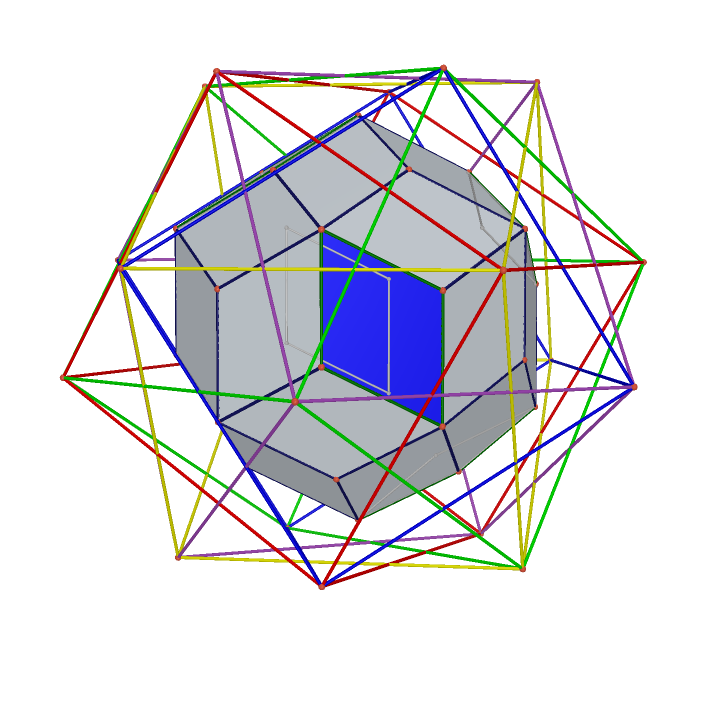Great rhombicuboctahedron Transparent great rhombicuboctahedron Small rhombicosidodecahedron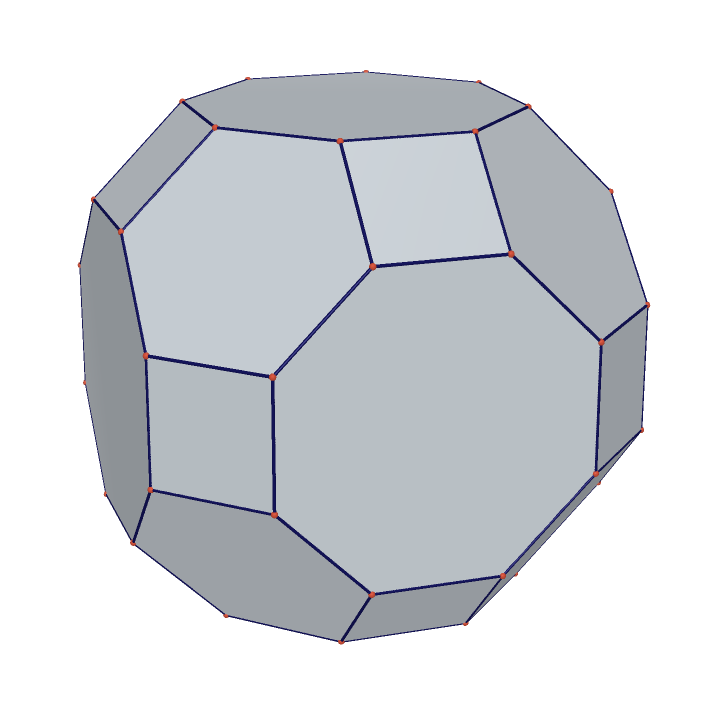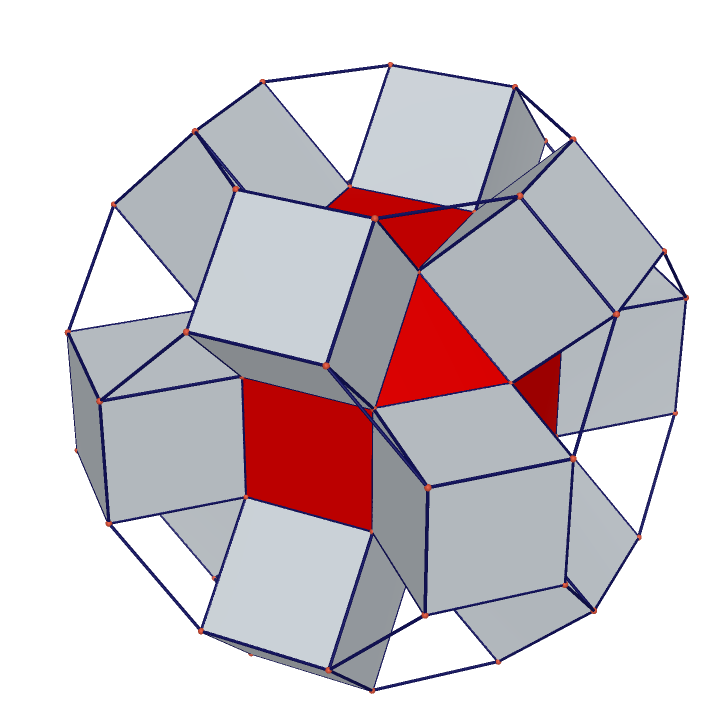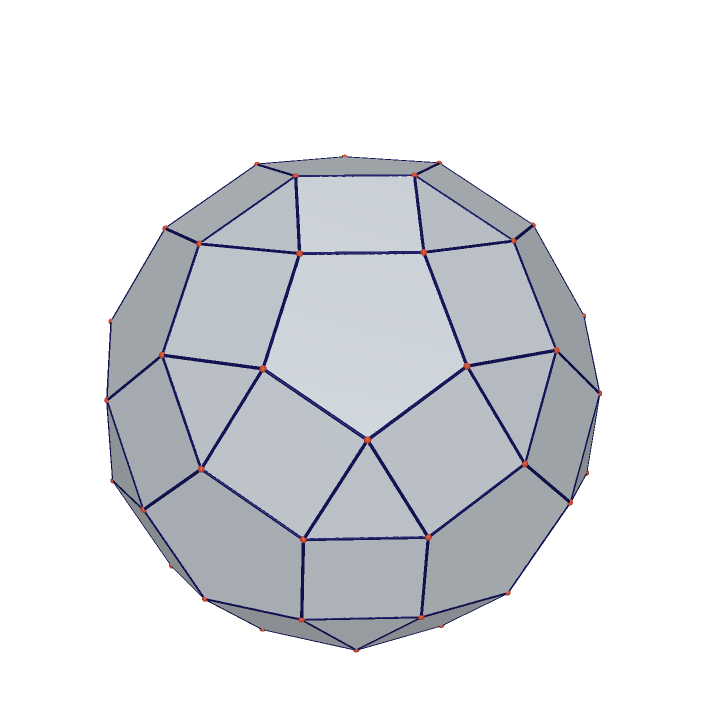Great rhombicosidodecahedron Deltoidal icositetrahedron Deltoidal hexecontahedron family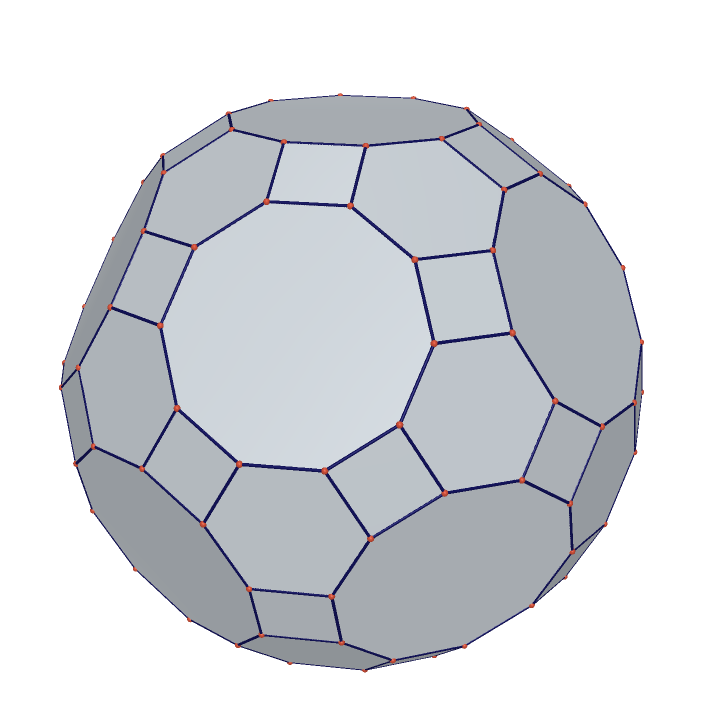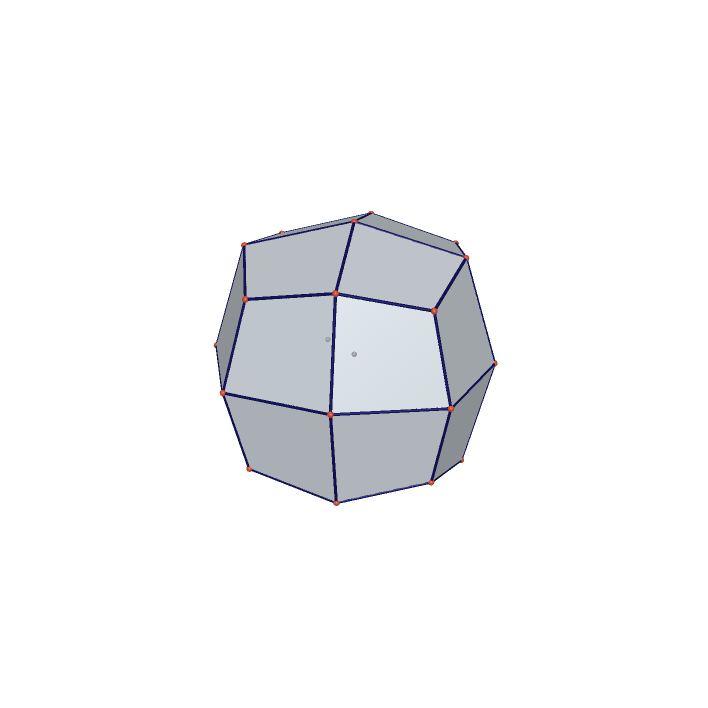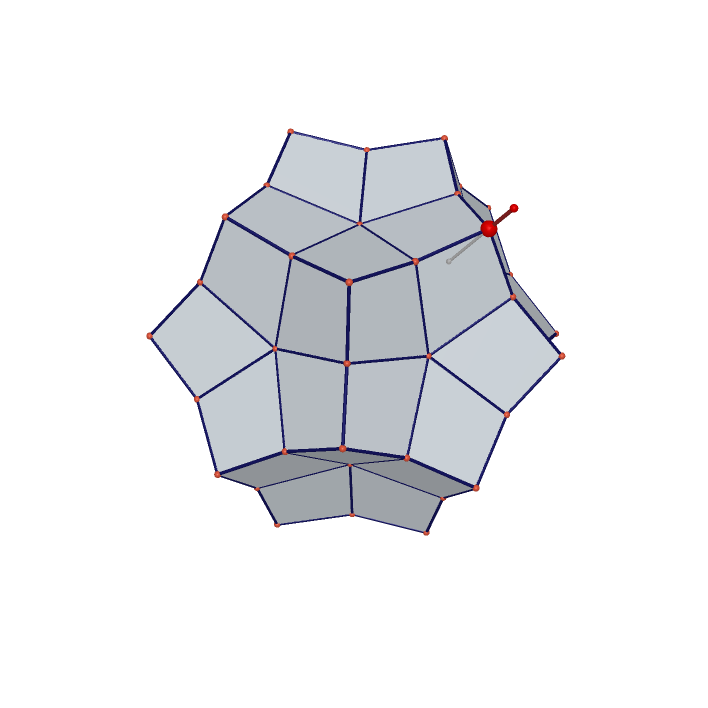The largest Icosahedron in Cube The smallest Cube containing Rhombic triacontahedron The largest Rhombic triacontahedron in Cube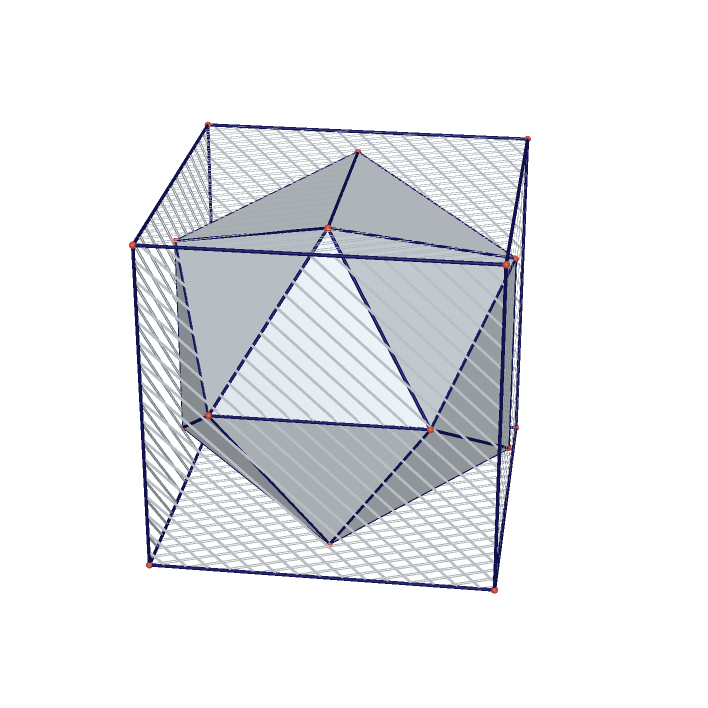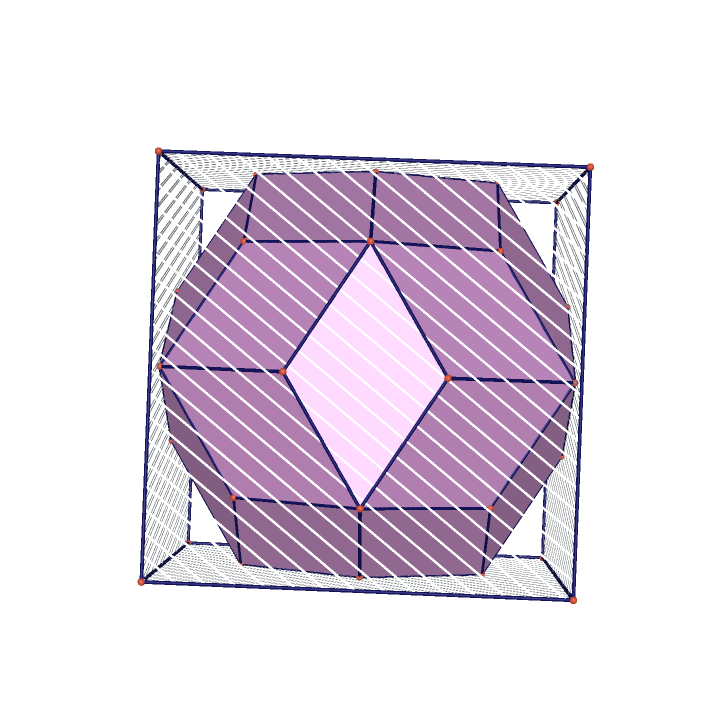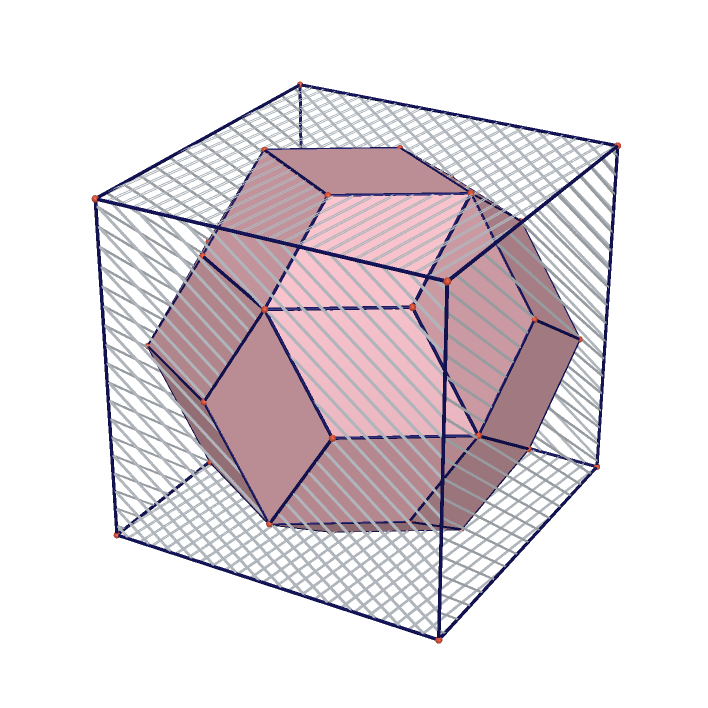Projection of Tetrahedron on Dodecahedron Projection of Tetrahedron on Dodecahedron Projection of Tetrahedron on Tetrahedron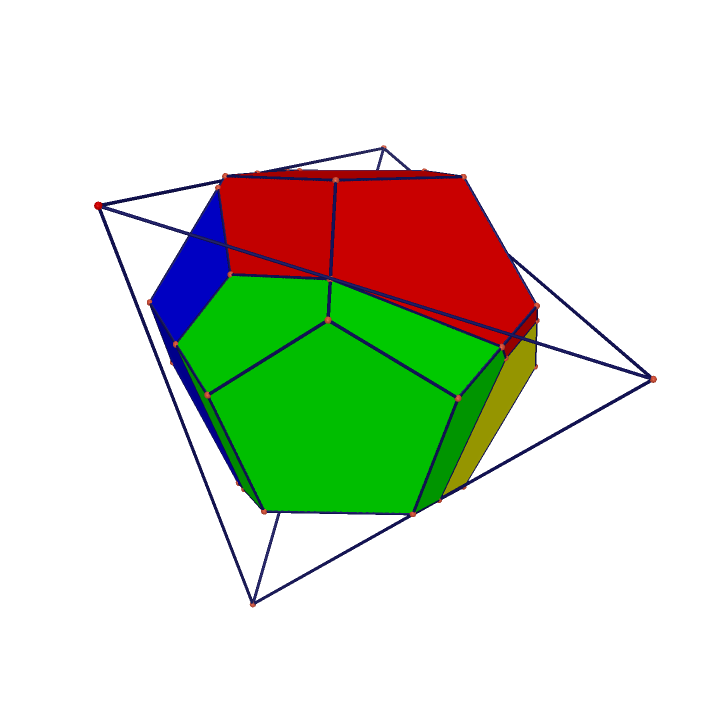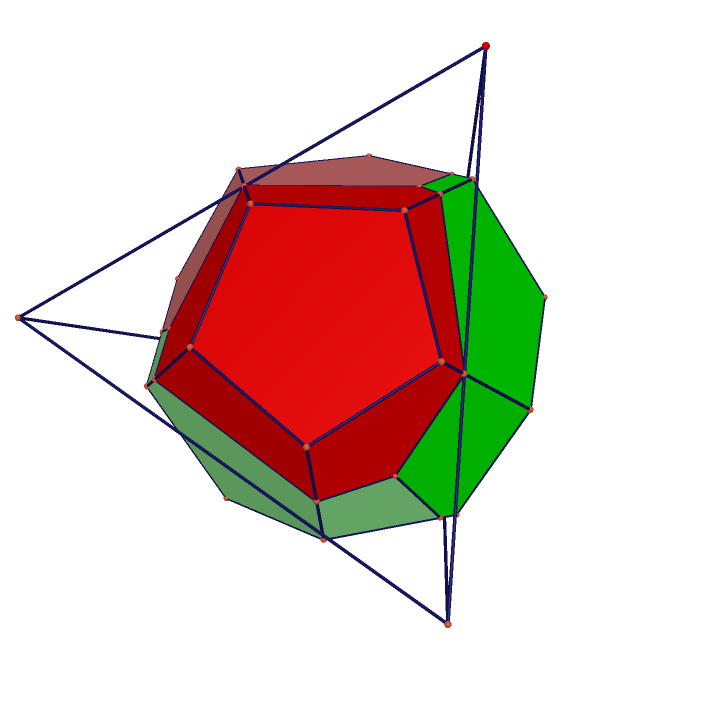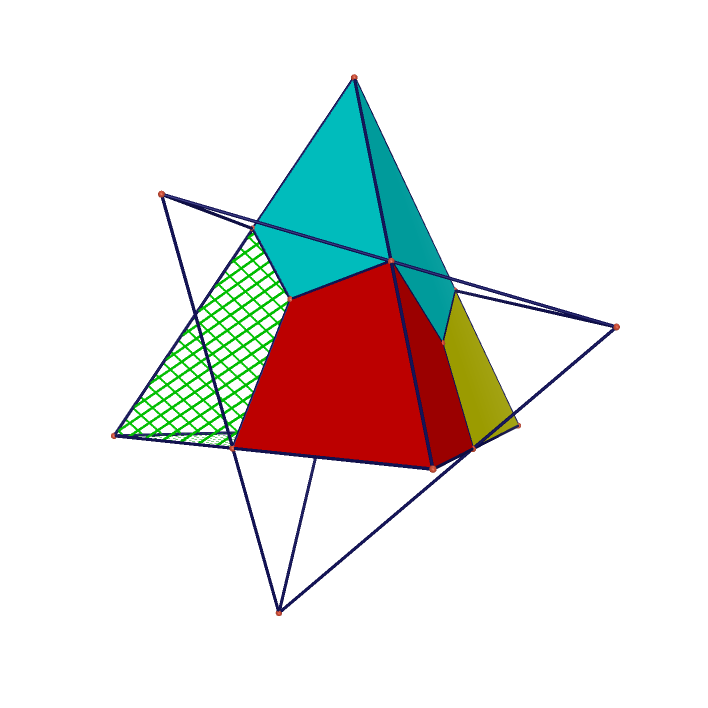Projection of Cube on Dodecahedron Projection of Cube on Octahedron Projection of Tetrahedron on Cube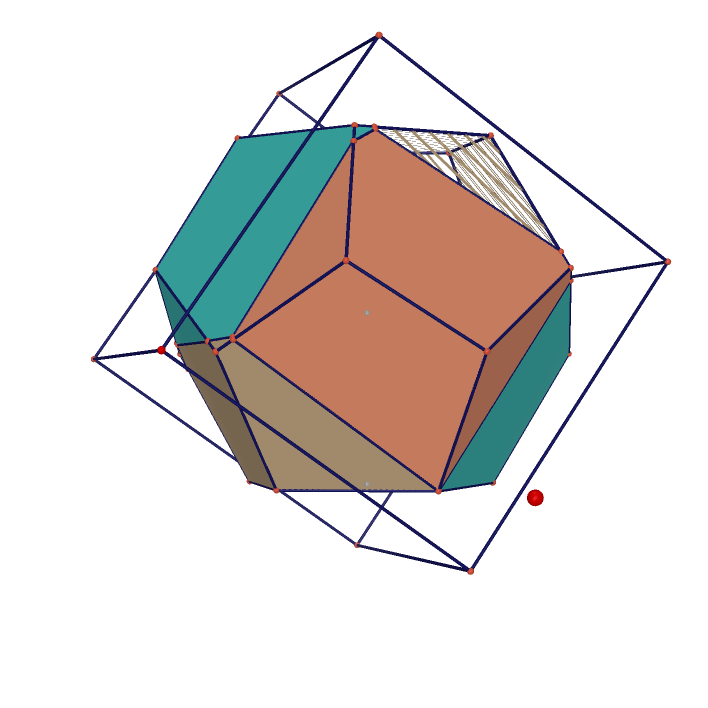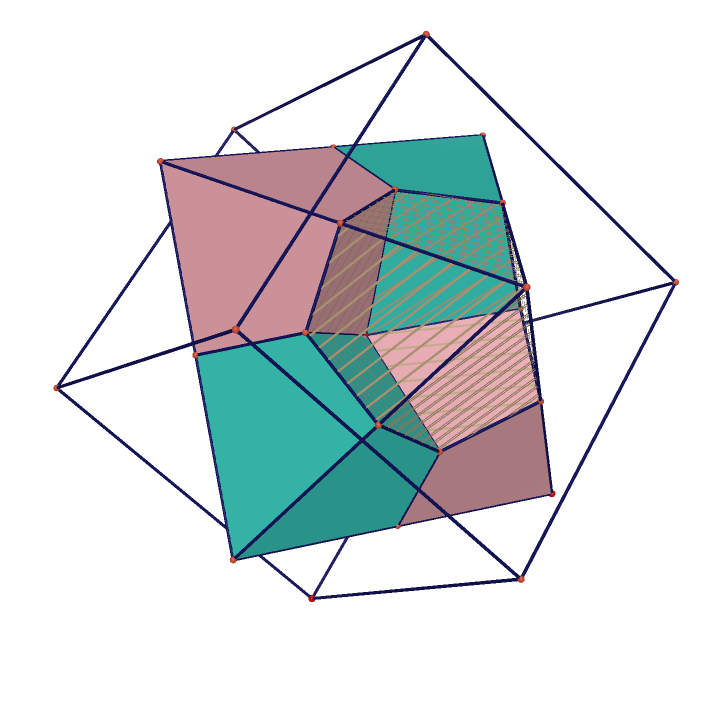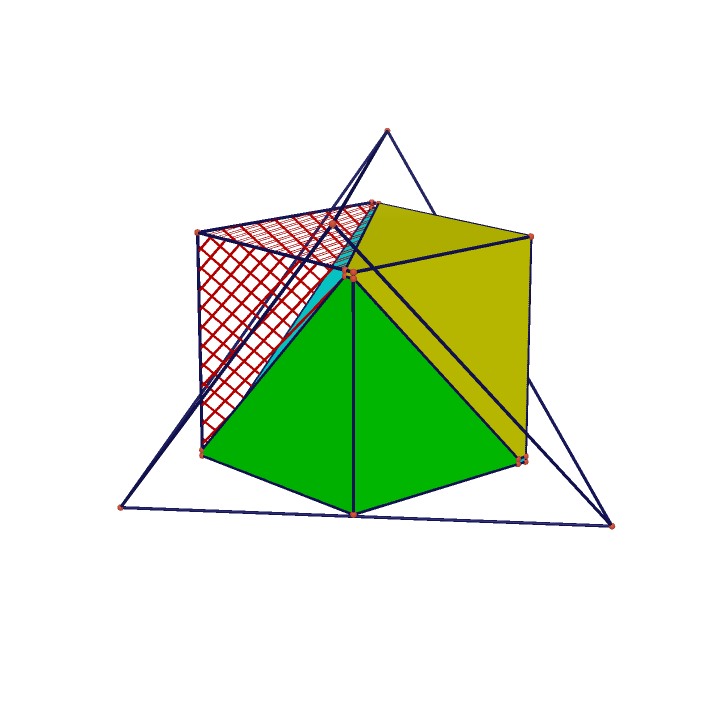The smallest Cube containing Octahedron The smallest Cube containing Rhombic Triacontahedron Projection of Cube on Cube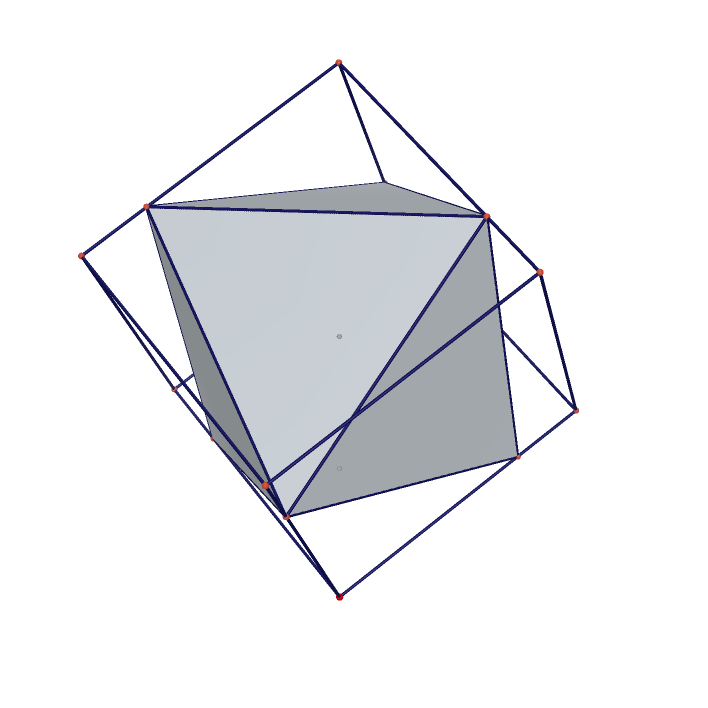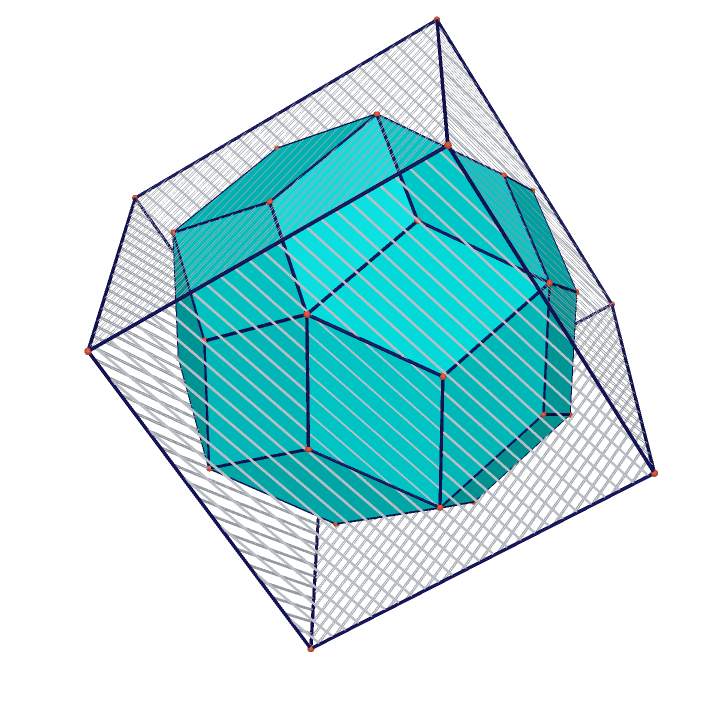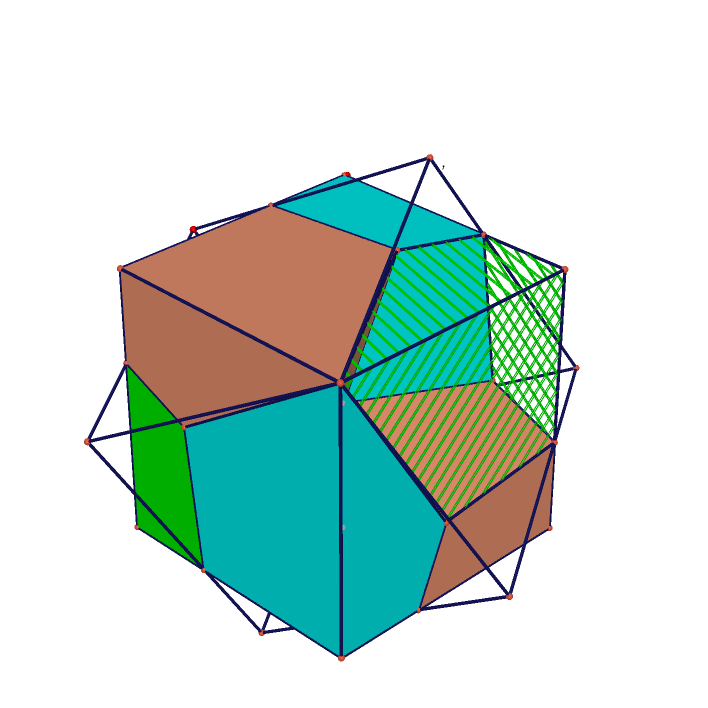The largest Icosahedron in Cube 2 The largest Octahedron in Cube The largest Rhombic Triacontahedron in Cube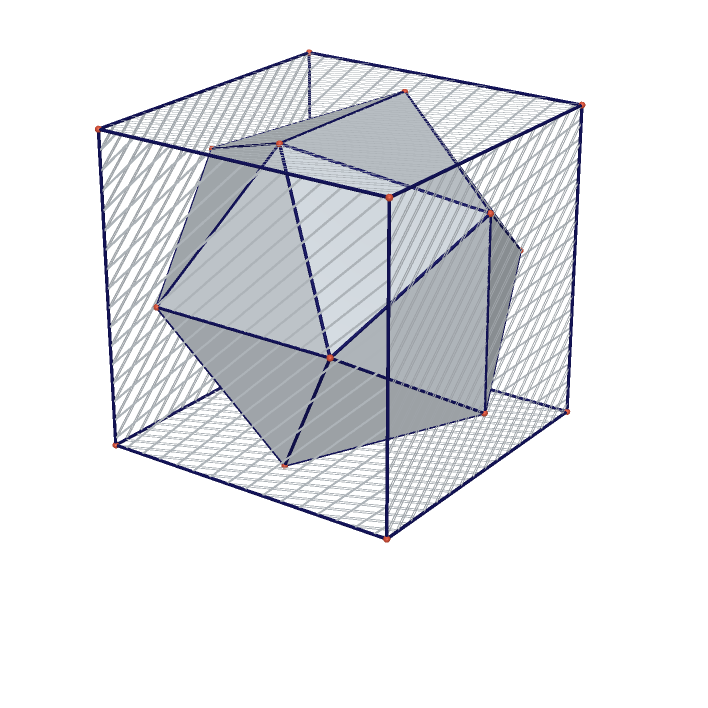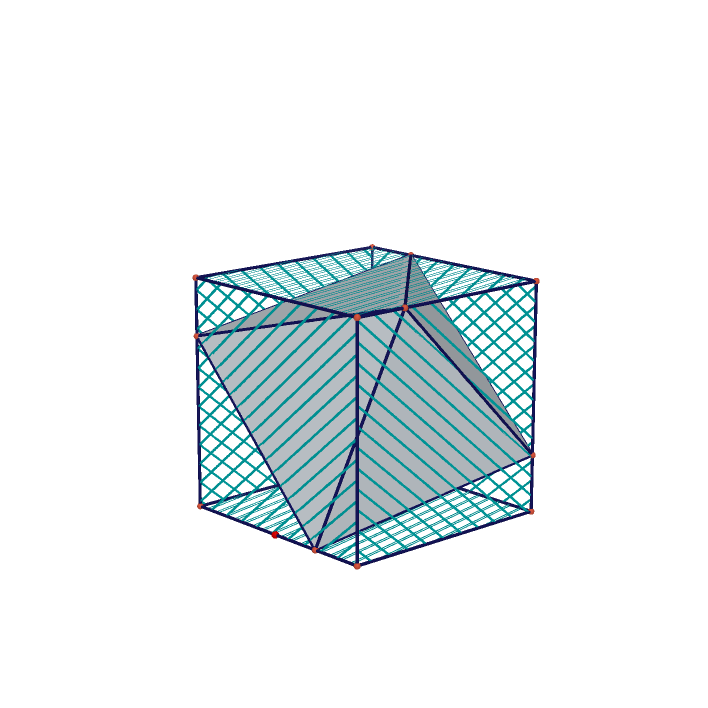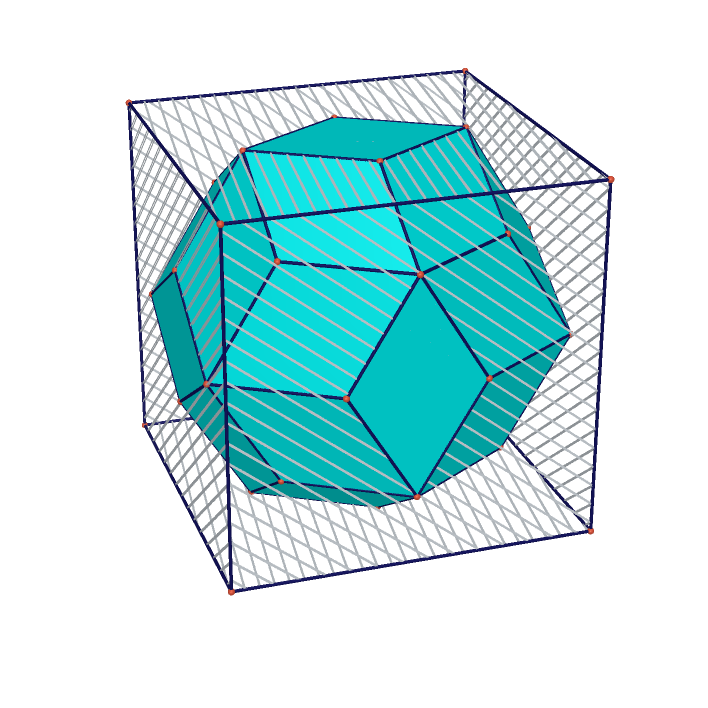The largest Dodecahedron in Cube 1 The largest Dodecahedron in Cube 2 The largest Icosahedron in Cube 1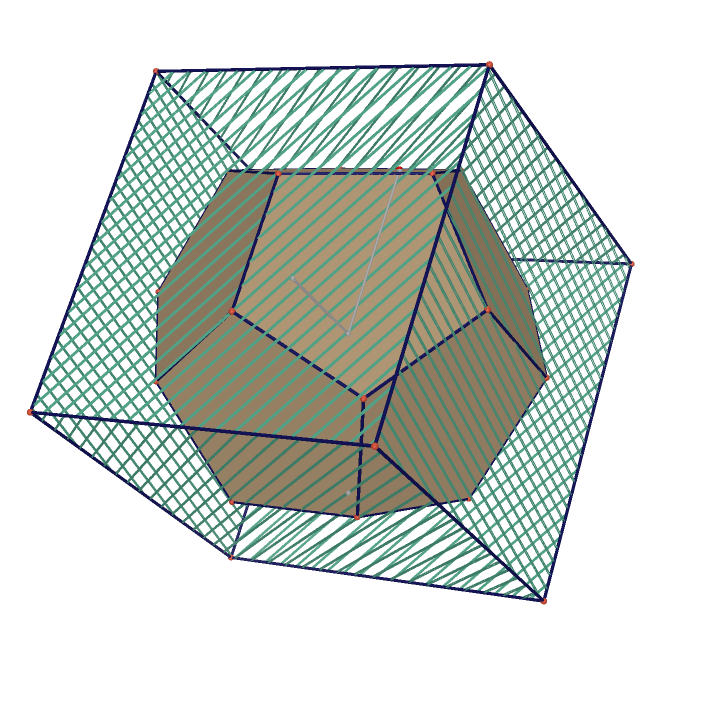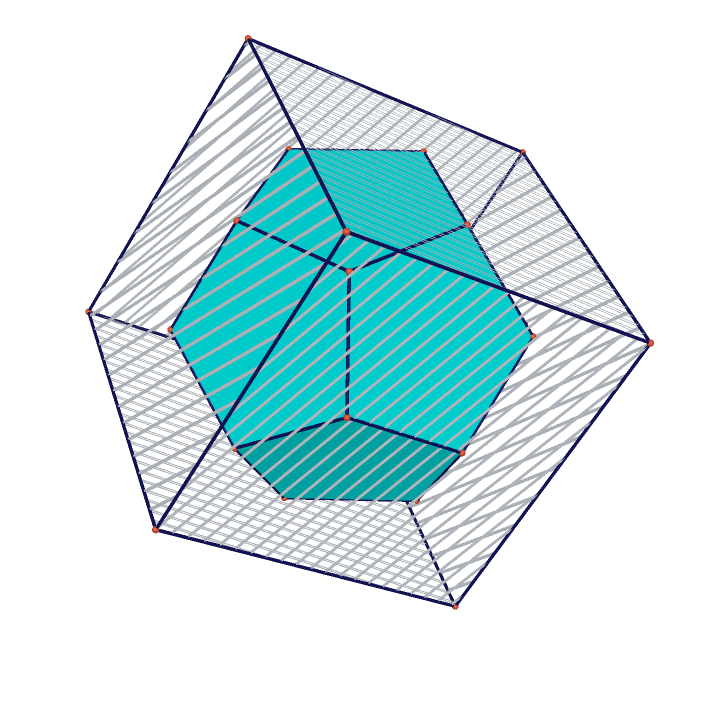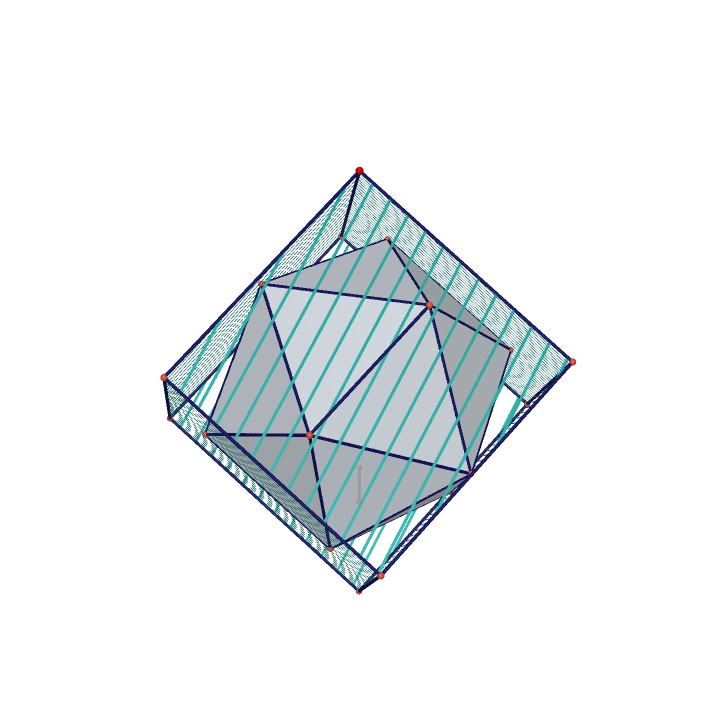30 tangent cylinders 30 pentagon pencils 12 sticks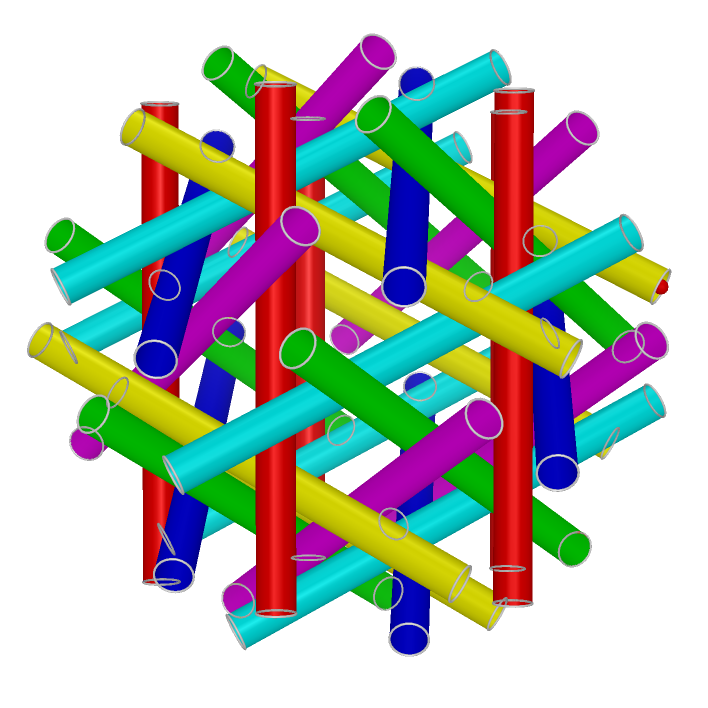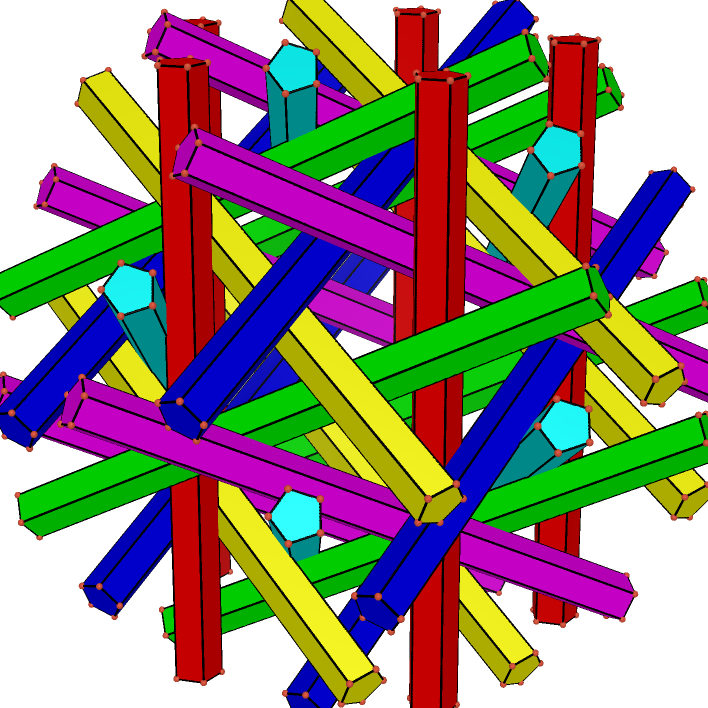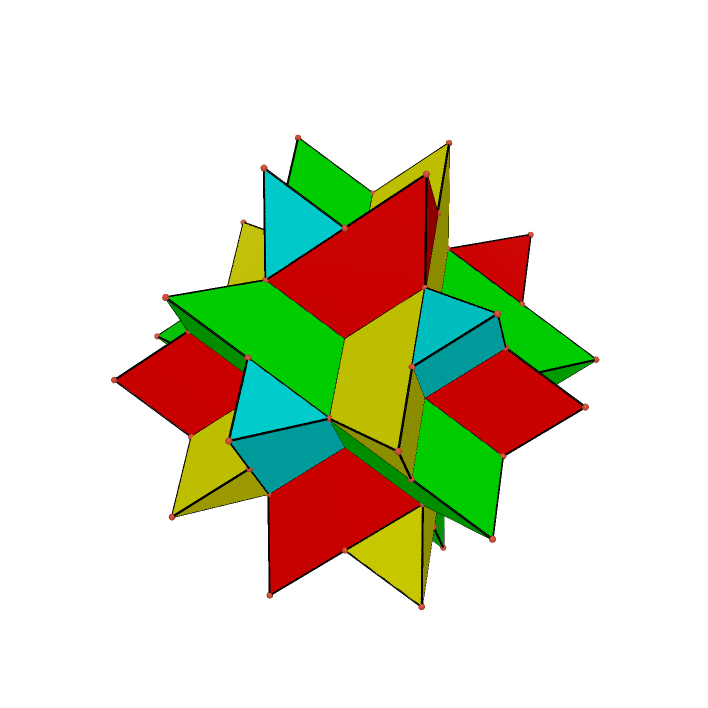Net of Icosidodecahedron Moving Deltoidal Icositetrahedron and 6 Rhombic Hexahedrons Turn a Dodecahedron inside out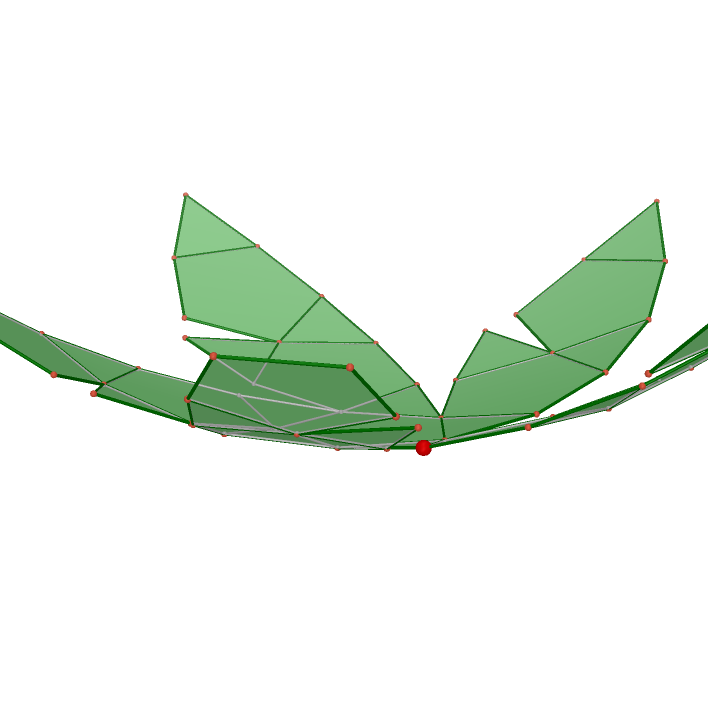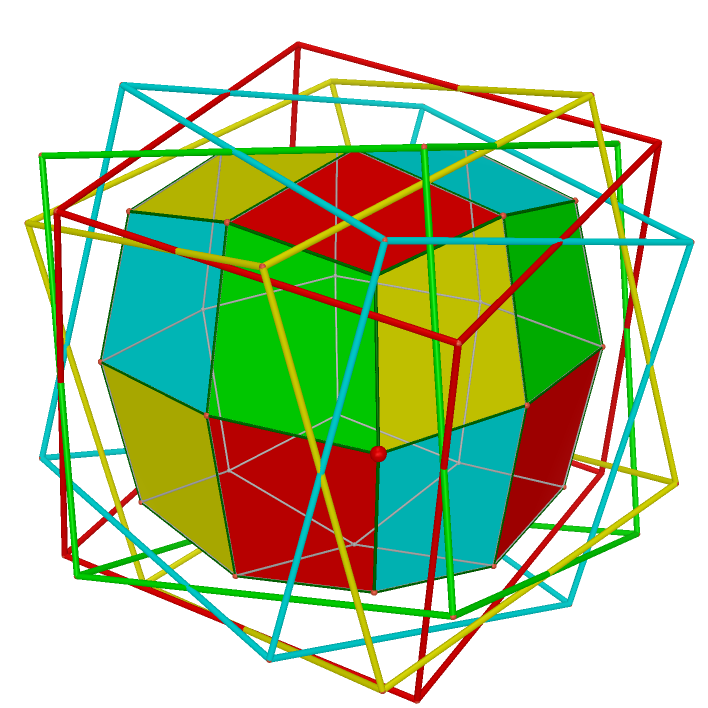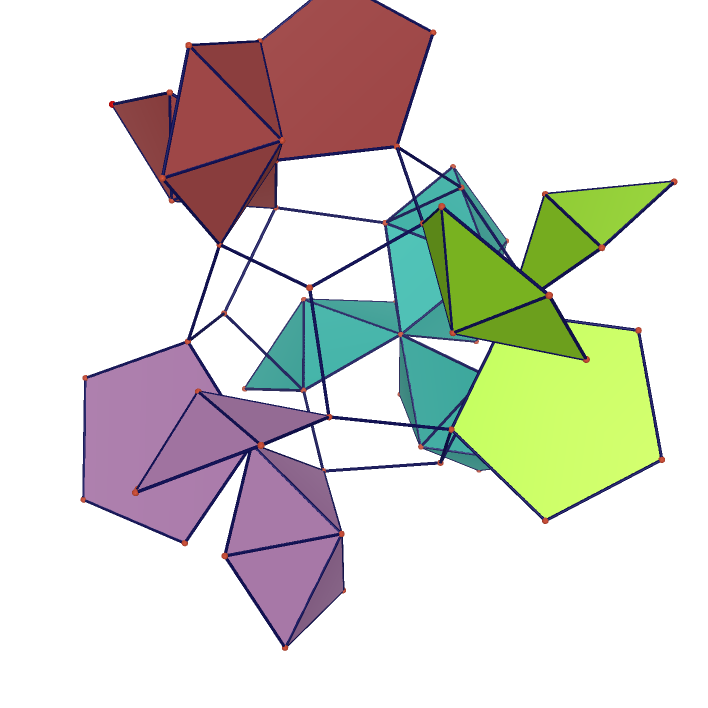Turn a Dodecahedron inside out 2 Net of Cube Net of Dodecahedron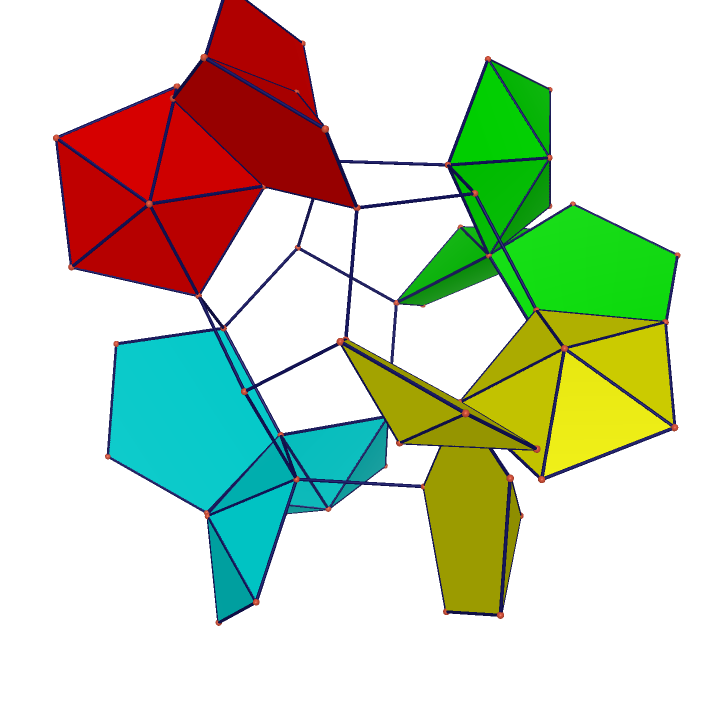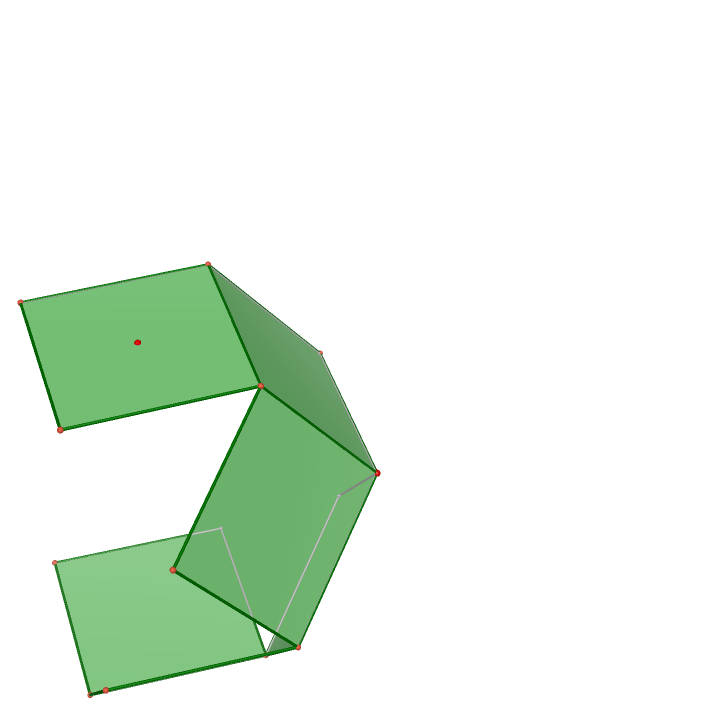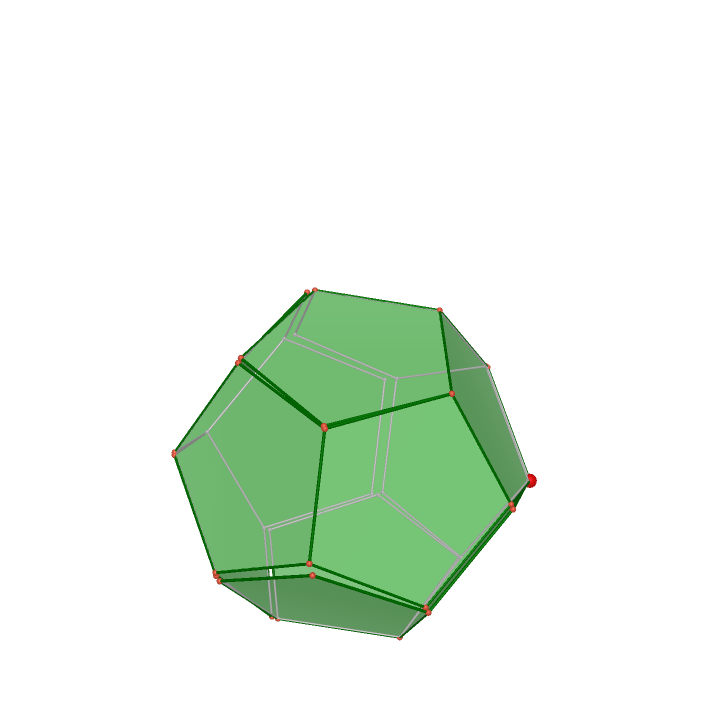The intersection of 5 Octahedron(Icosahedron) The intersection of 5 Tetrahedrons(Icosahedron) The intersection of the 5 Cubes(Rhombic triacontahedron)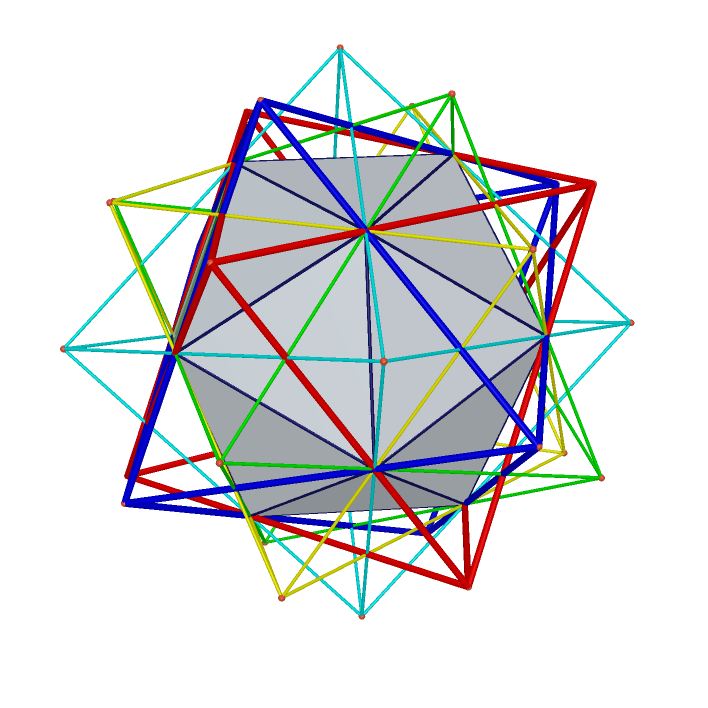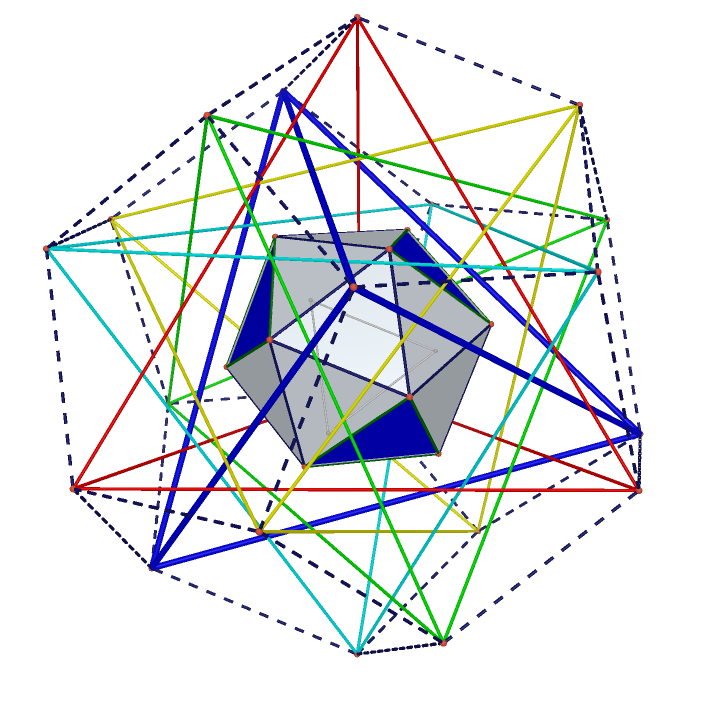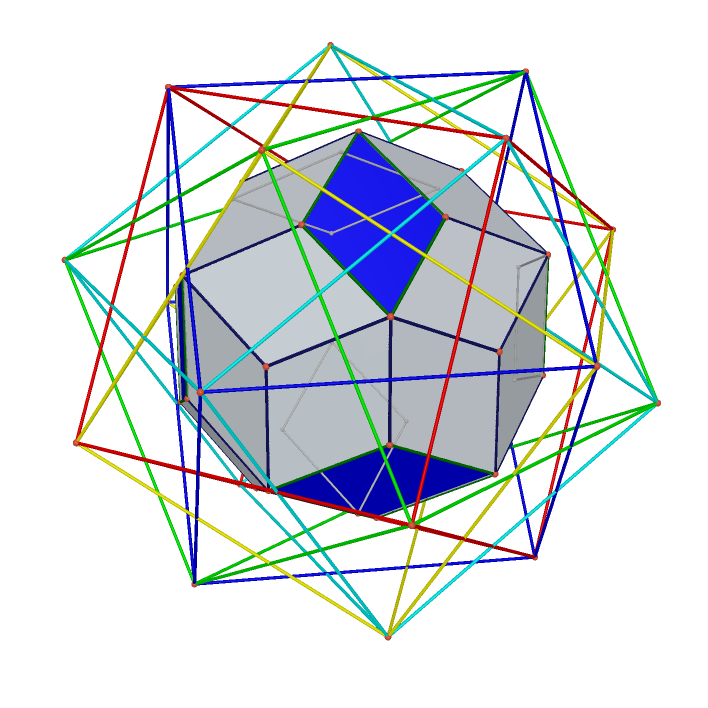Duality between Dodecahedron and Icosahedron Duality between Octahedron and Cube Relation between Buckyball Dodecahedron and Icosahedron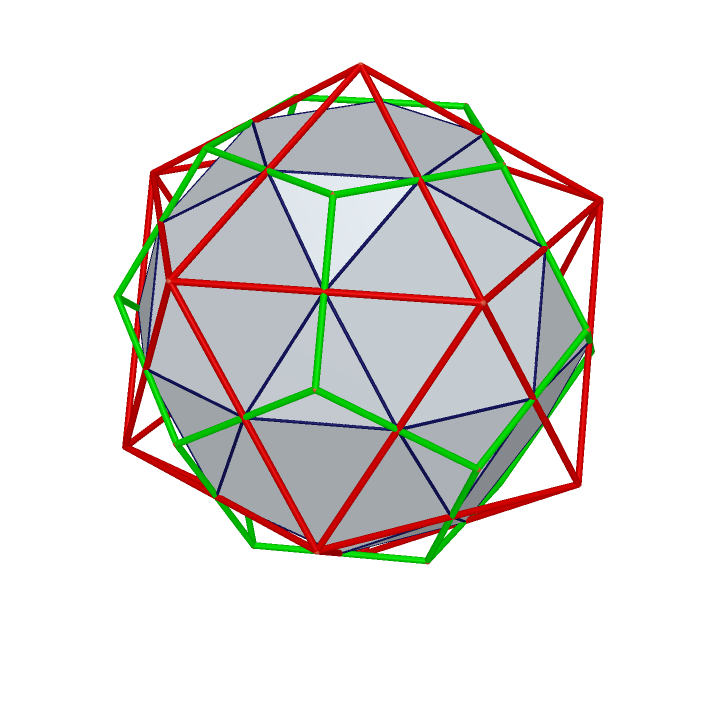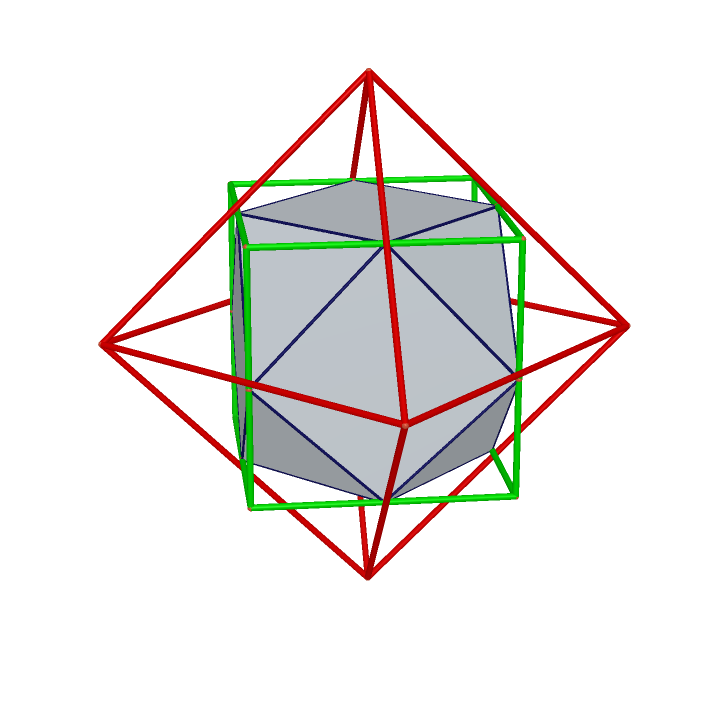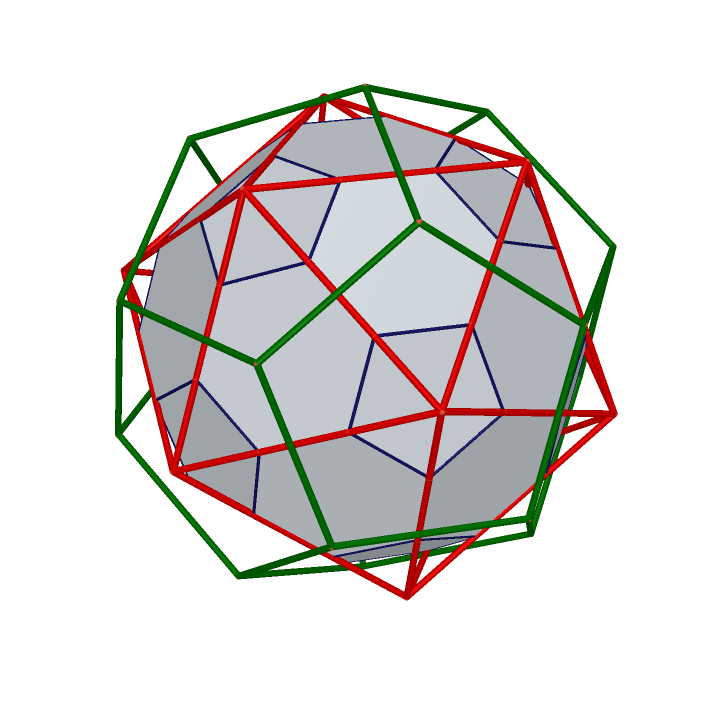The intersection of Cube Octahedron and Rhombic Dodecahedron(Great Rhombicuboctahedron) The intersection of 4 Rhombic Hexahedrons(Deltoidal icositetrahedron) Burrs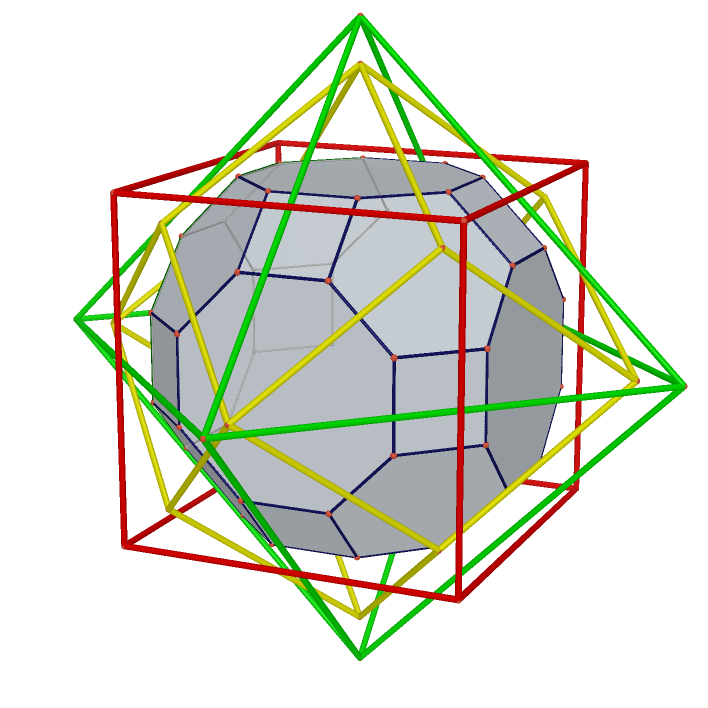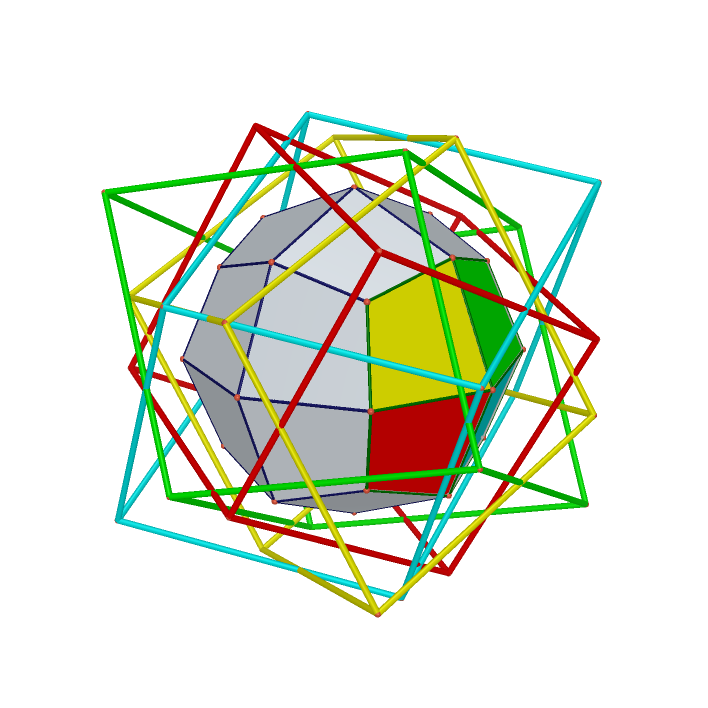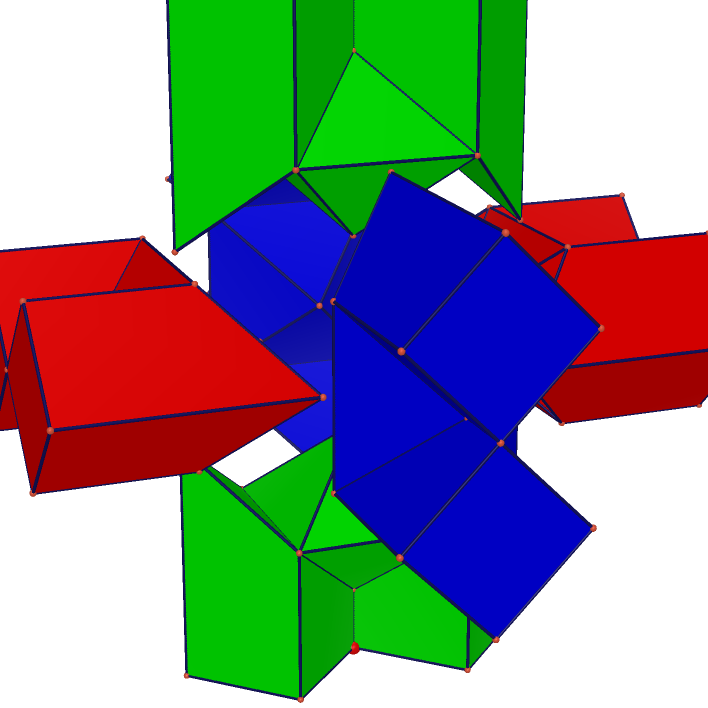Saddle Villarceau circles Twisted prismatic ring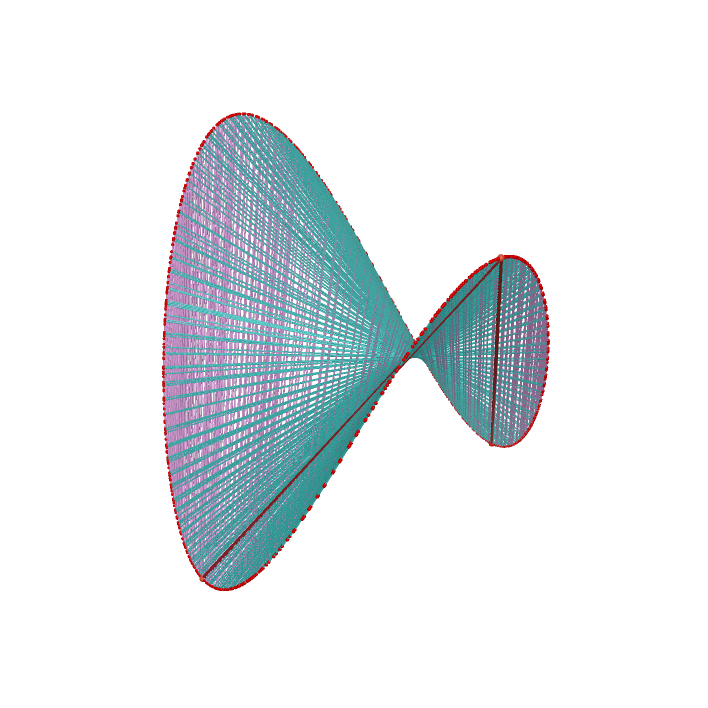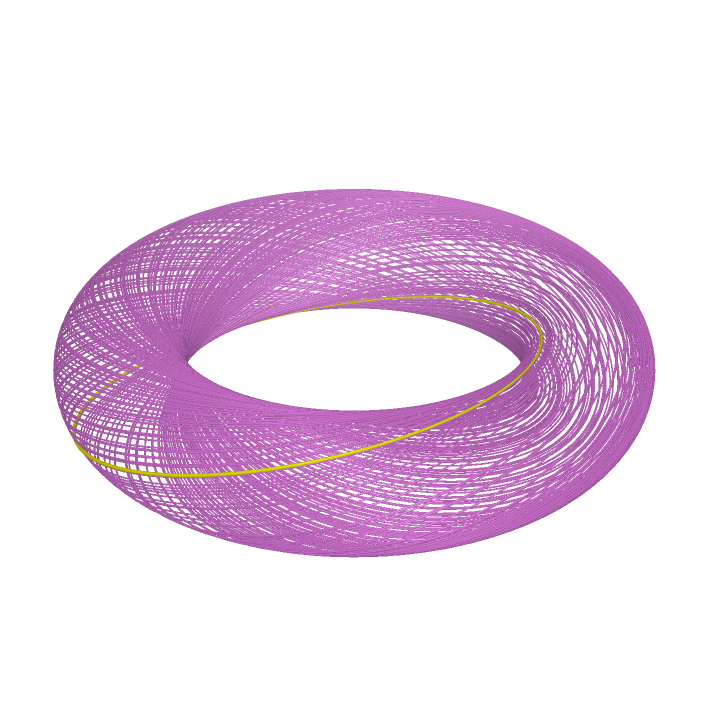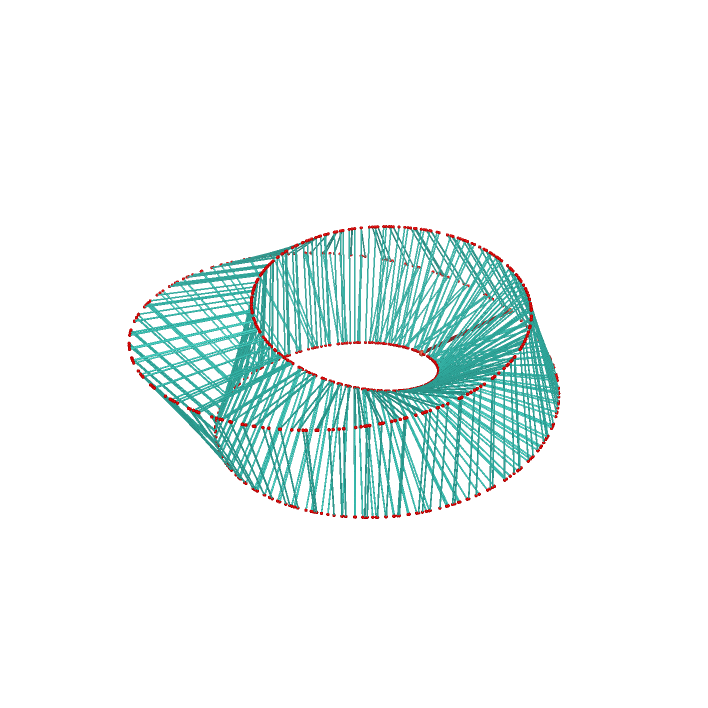Hexecontahedrons Inversions of Incircles of Faces of Trianglular Cupola Inversion Related to Dodecahedron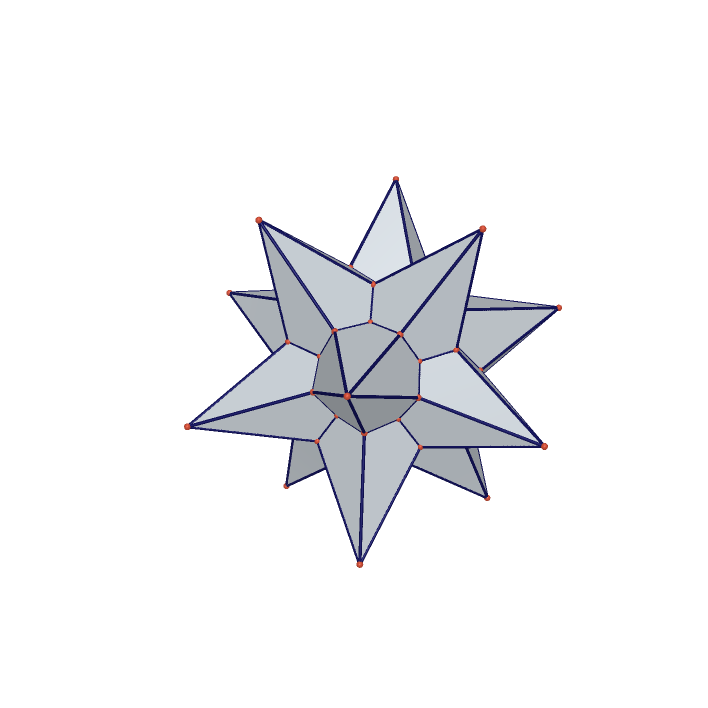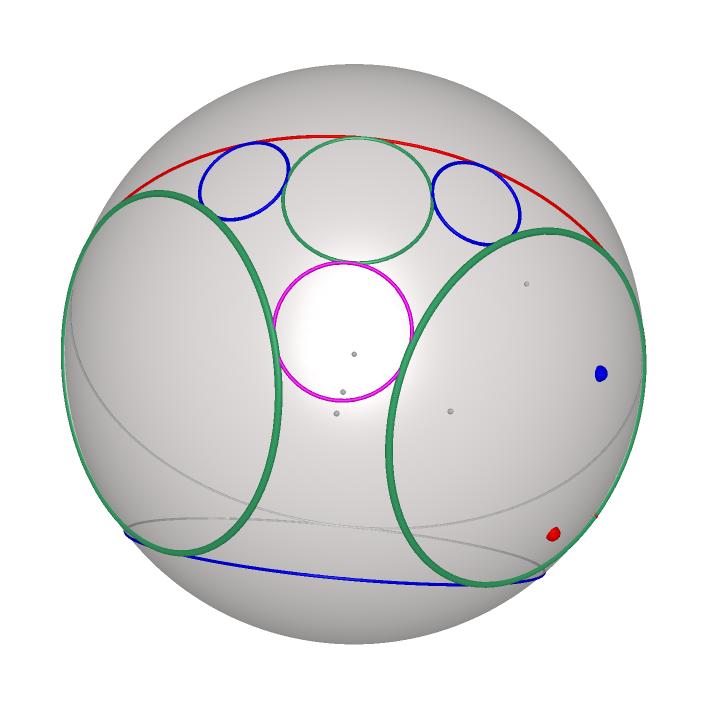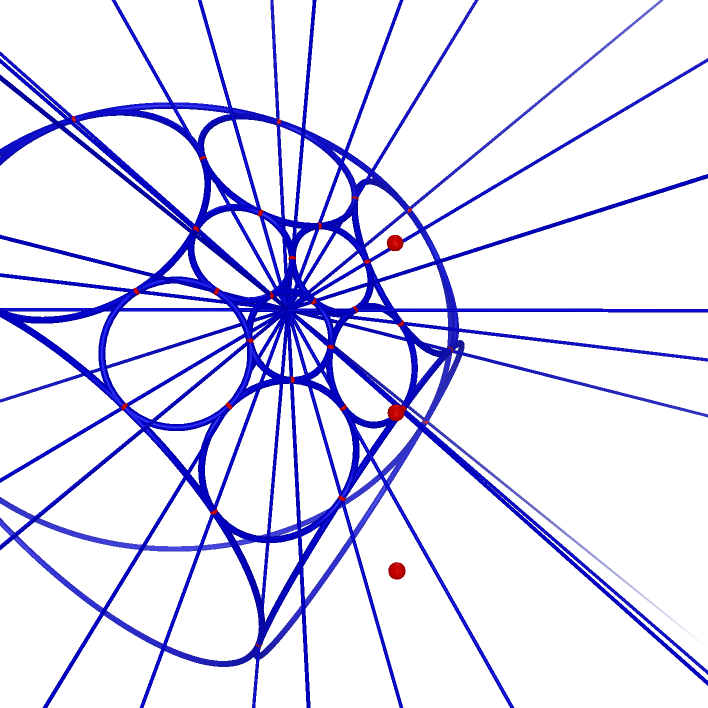Inversion of Inscribed Circles of Faces of Triangular Orthobicupola Inversion of Inscribed Circles of Faces of Triangular Orthobicupola Inspired by Paper Folding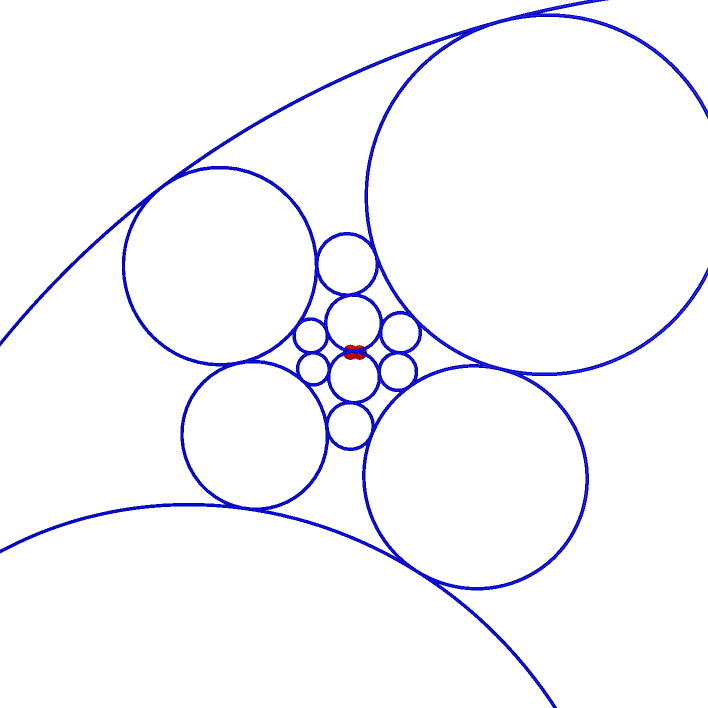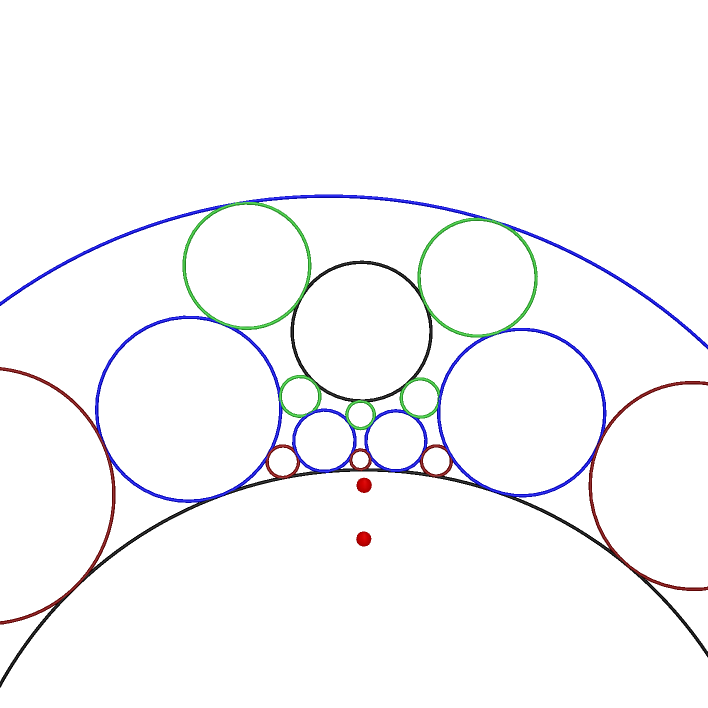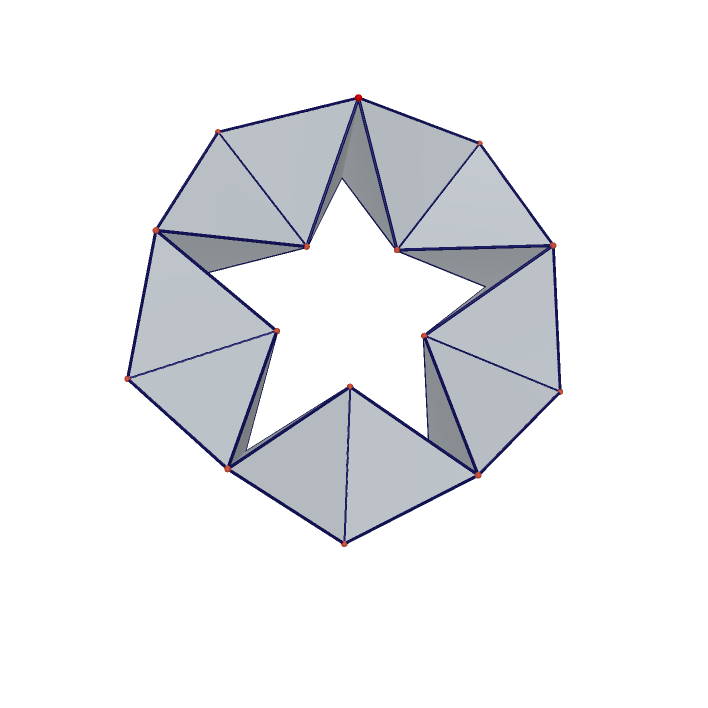180-Parallelogram-Faced Polyhedron A Chain of Four Circles Each Tangent to Two Fixed Intersection Circles Asymmetric Design Based on Triangular Cupola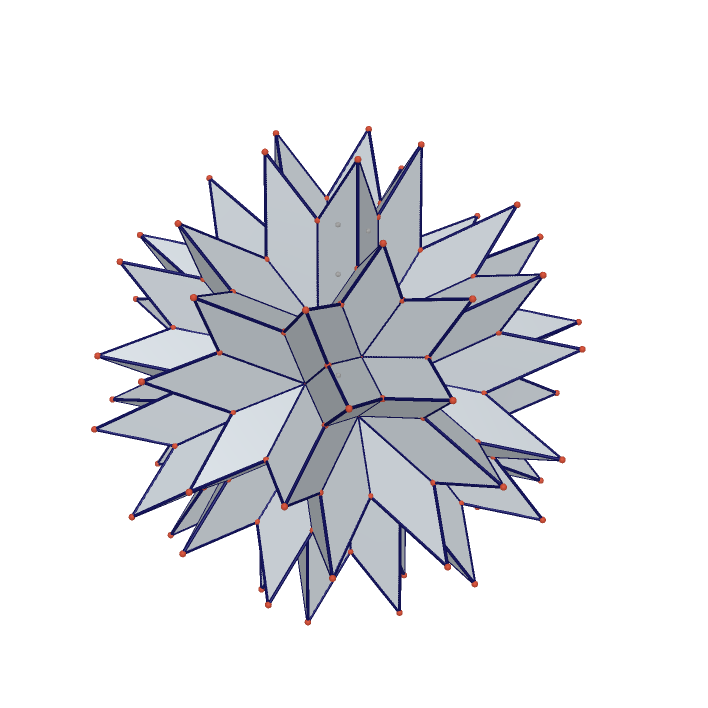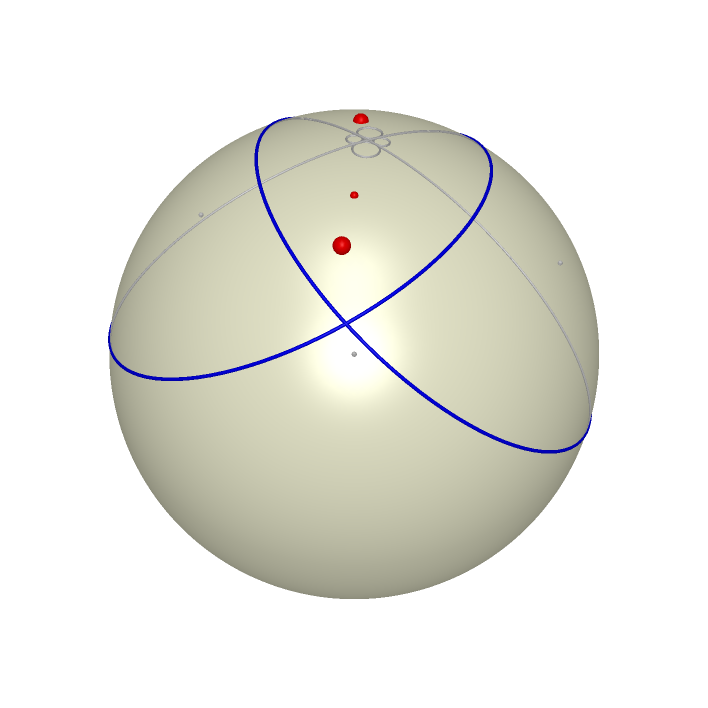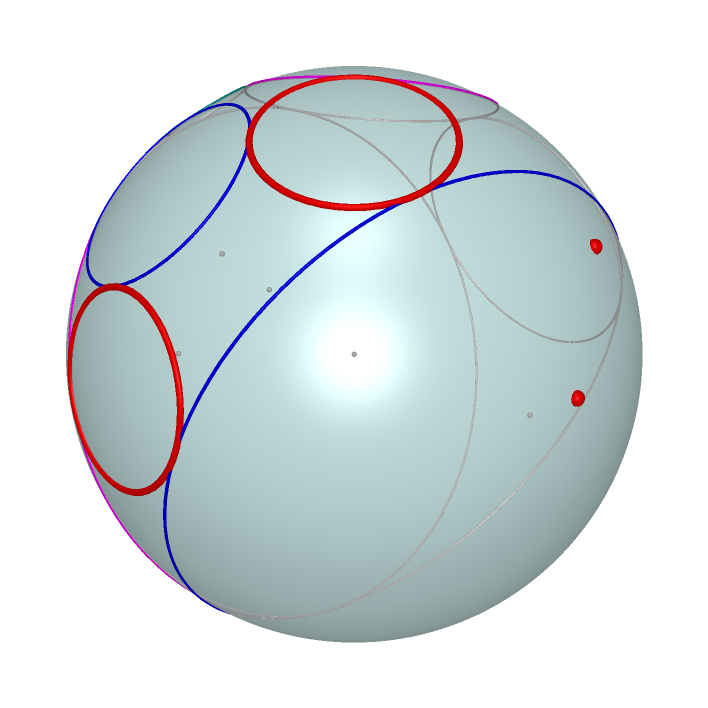Cube Projected on Bucky Ball Four Circles Each Common Tangent to Two Fixed Circles and Their Stereographic Images Maximal Kaleidocycle formed by 10 Tetrahedrons